# NCERT Exemplar Solutions for Class 7 Maths Chapter 9 Perimeter and Area

NCERT Exemplar Solutions for Class 7 Maths Chapter 9 Perimeter and Area are provided here. To cover the entire syllabus in Maths, the NCERT Exemplar is an essential material as it offers a wide range of questions that test the students’ understanding of concepts. Our expert personnel have solved the problems step by step with neat explanations. Students who aspire to score good marks in Maths, then refer to NCERT Exemplar Solutions for Class 7 Maths.

Perimeter is the distance around a closed figure, while area is the part of a plane or region occupied by a closed figure. Now, let us have a look at some of the concepts discussed in this Chapter.

• Squares and Rectangles
• Triangles as Parts of Rectangles
• Area of a Parallelogram
• Area of Triangle
• Circumference of a Circle
• Area of Circle

## Download the PDF of NCERT Exemplar Solutions for Class 7 Maths Chapter 9 Perimeter and Area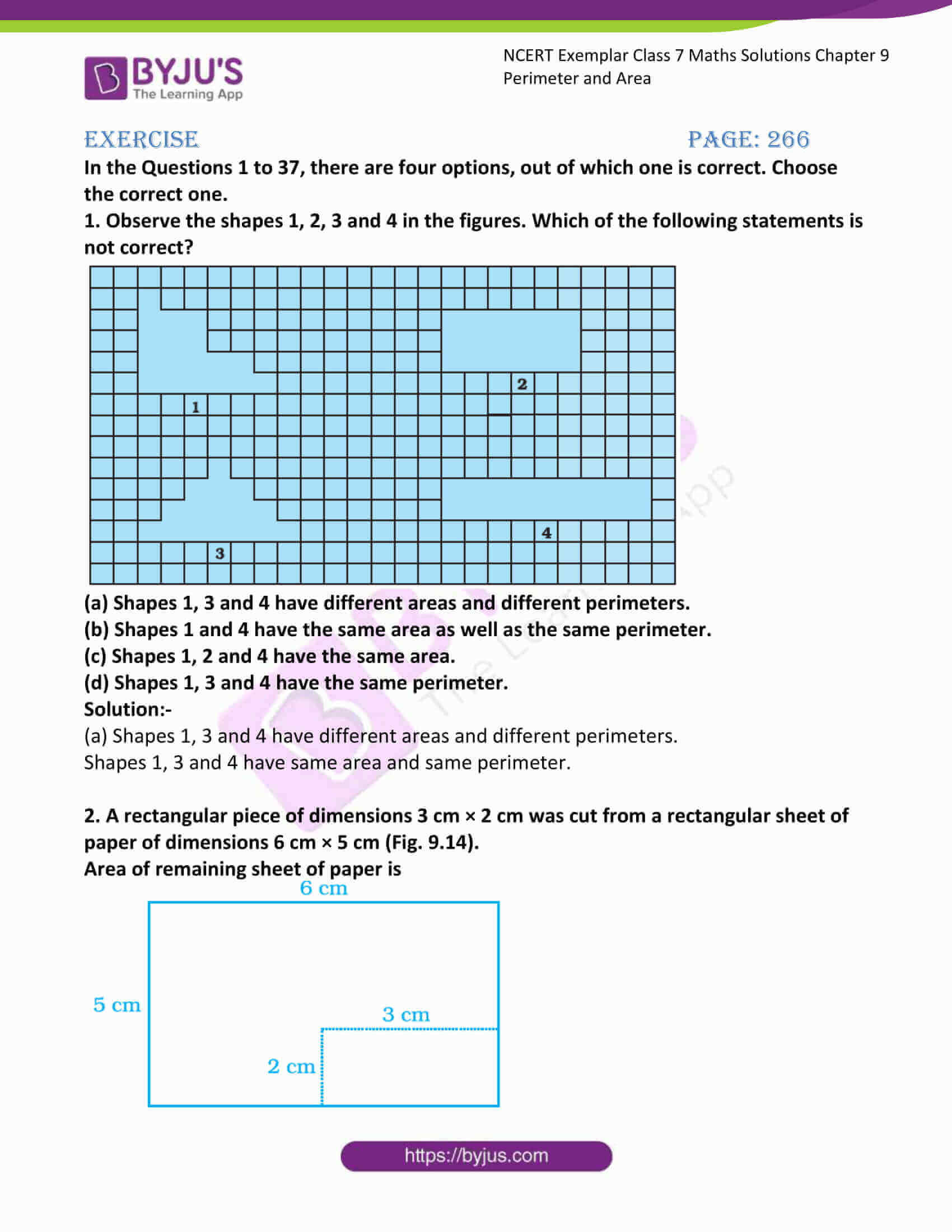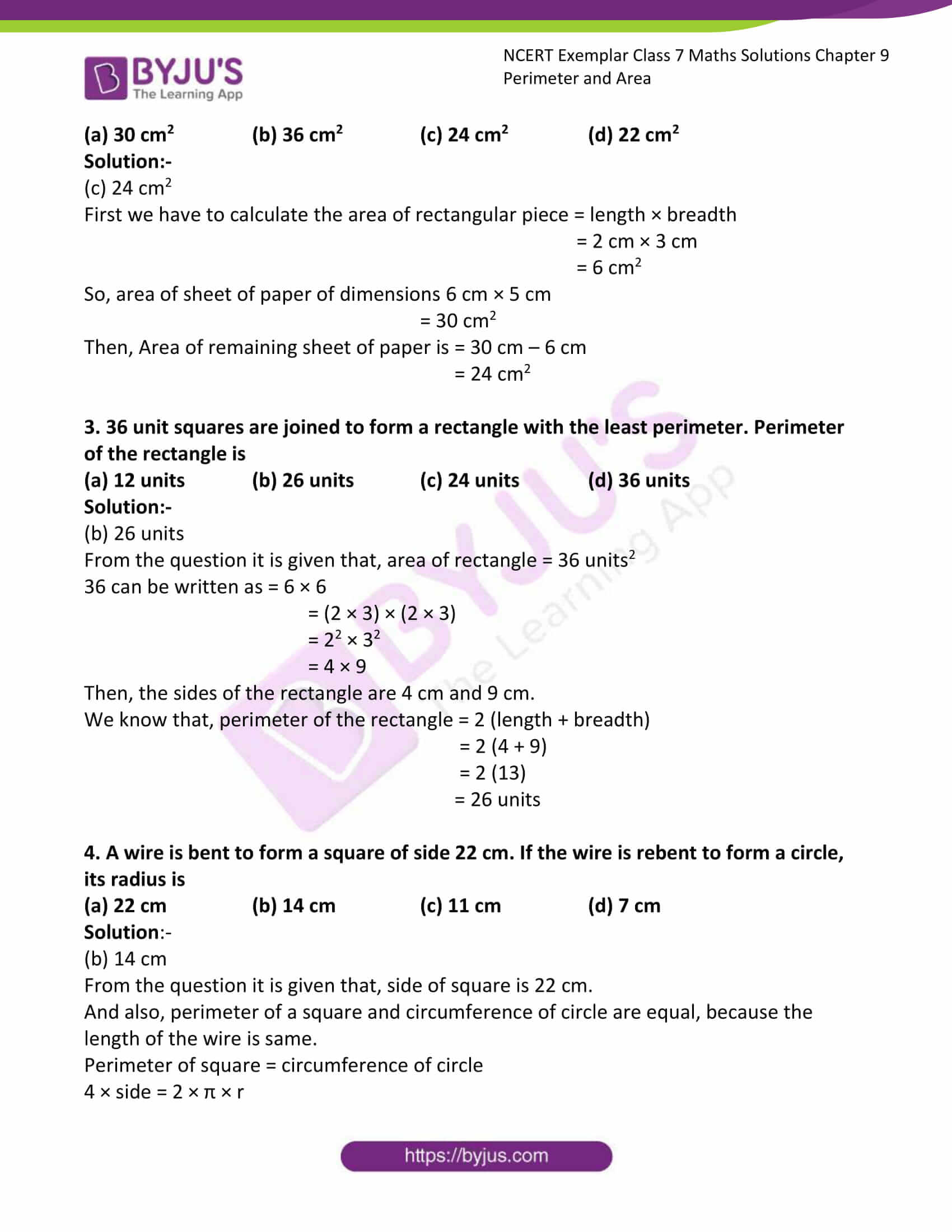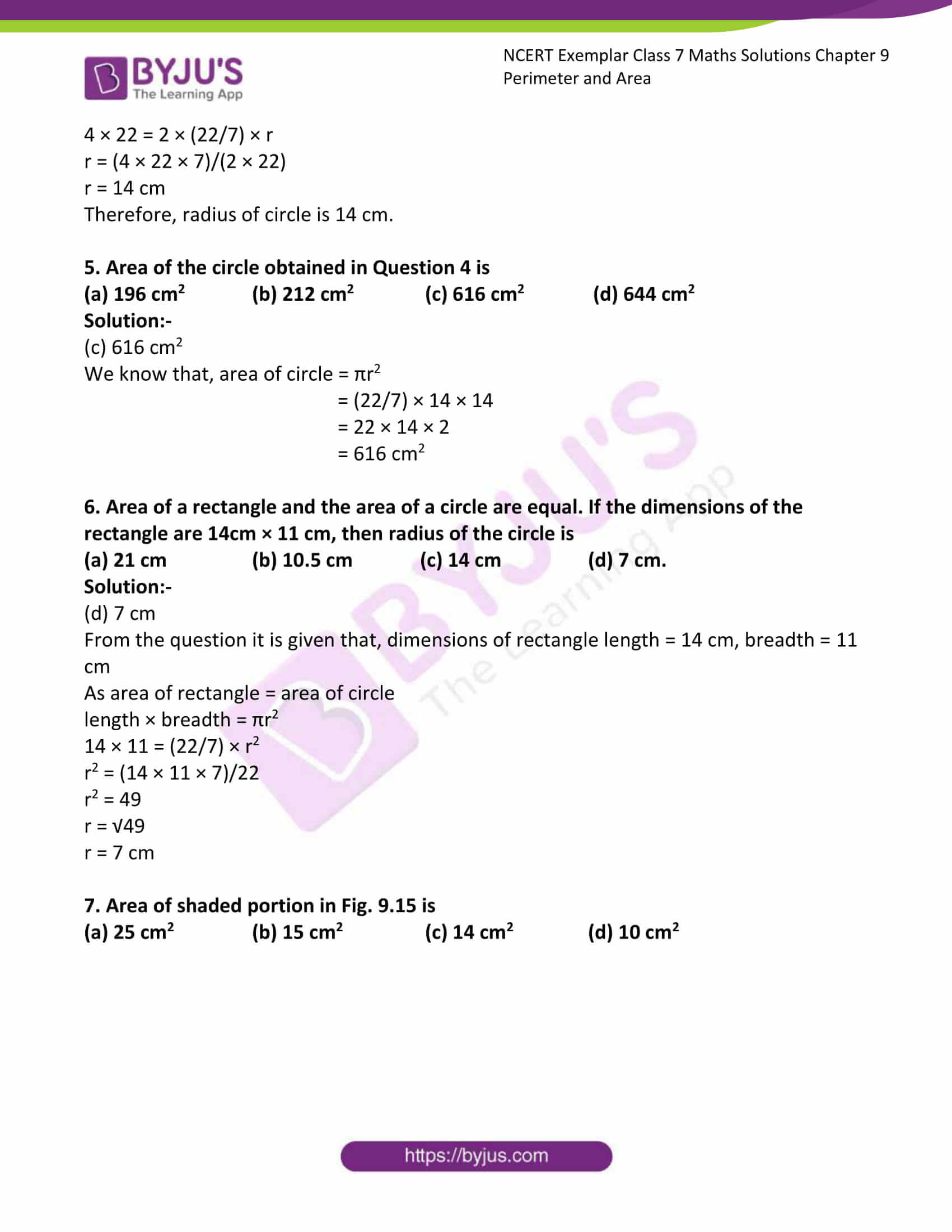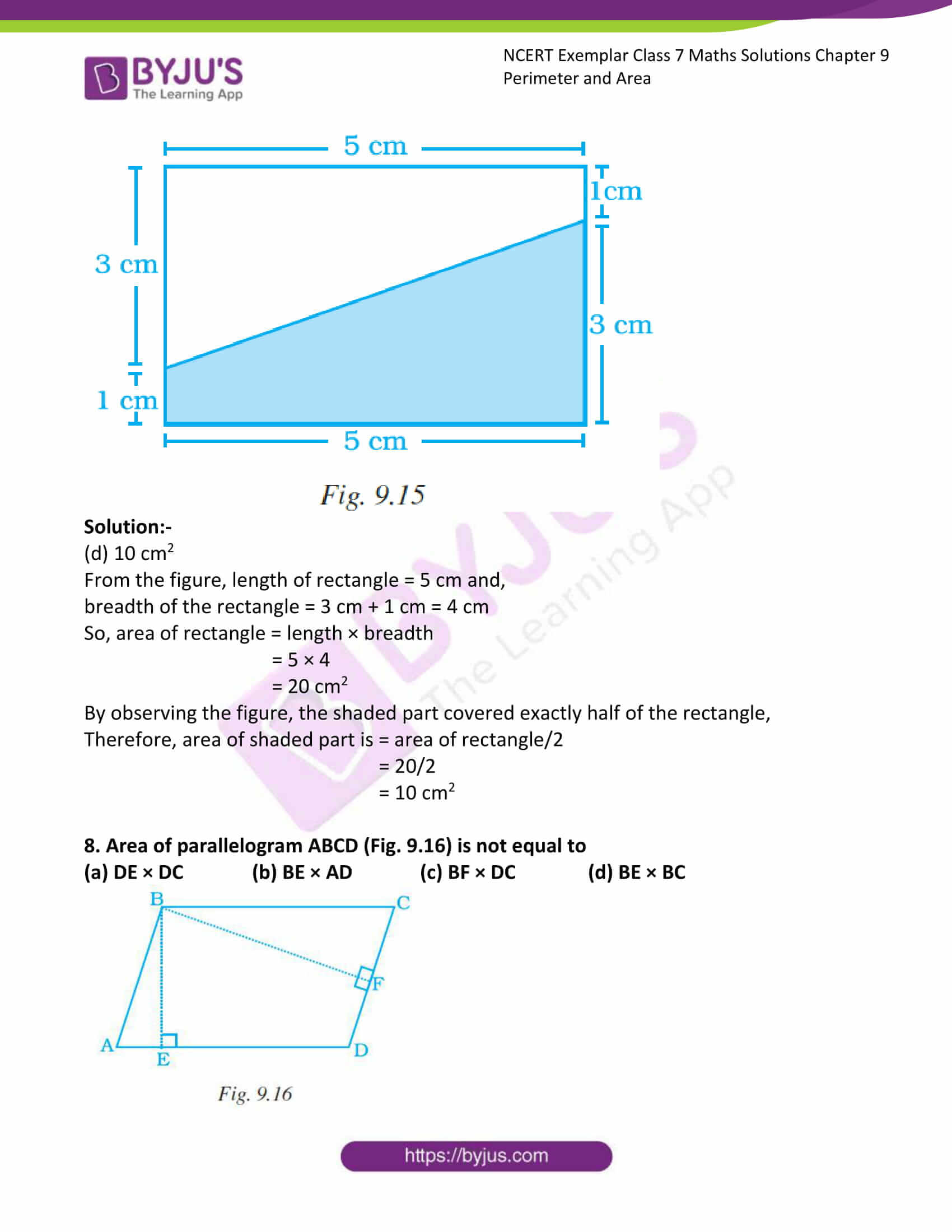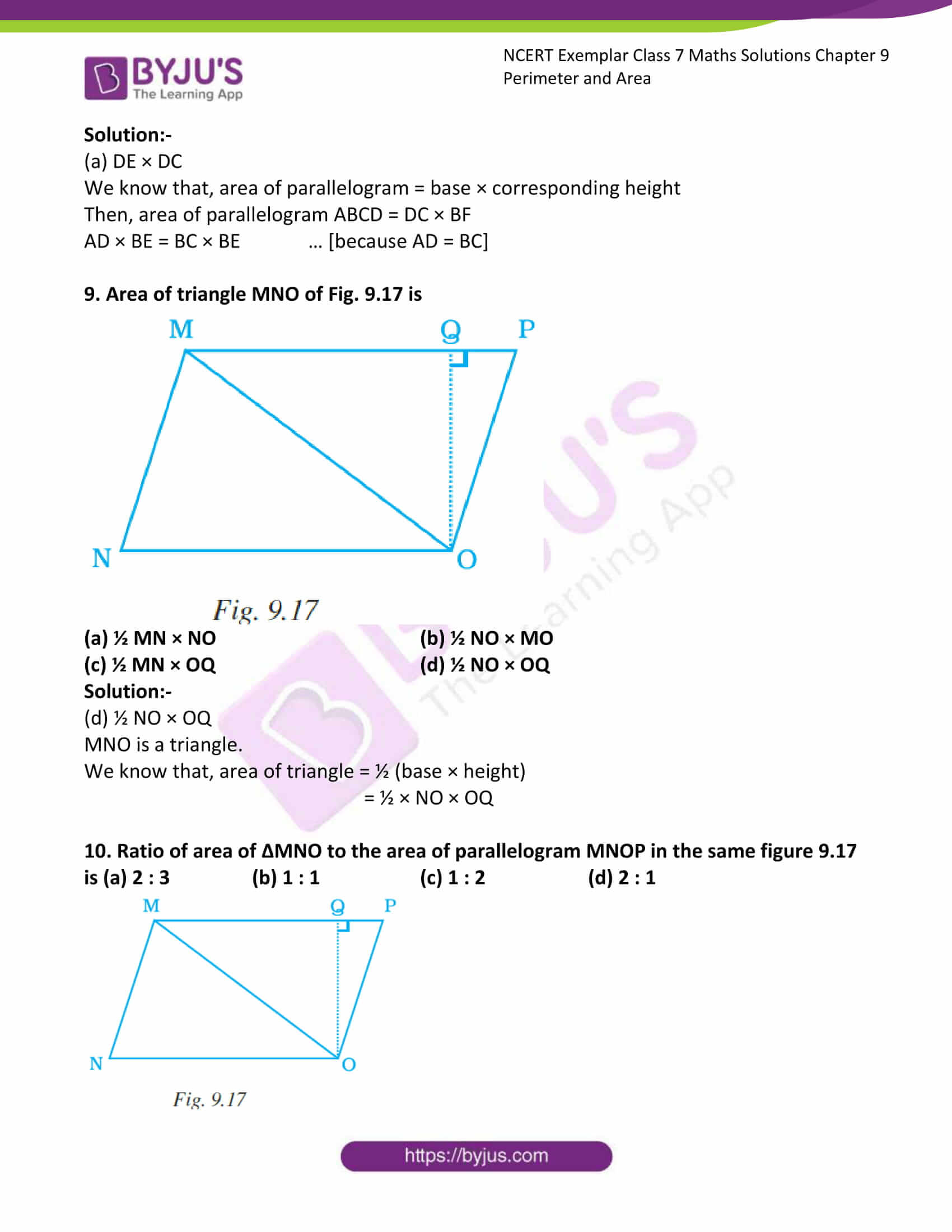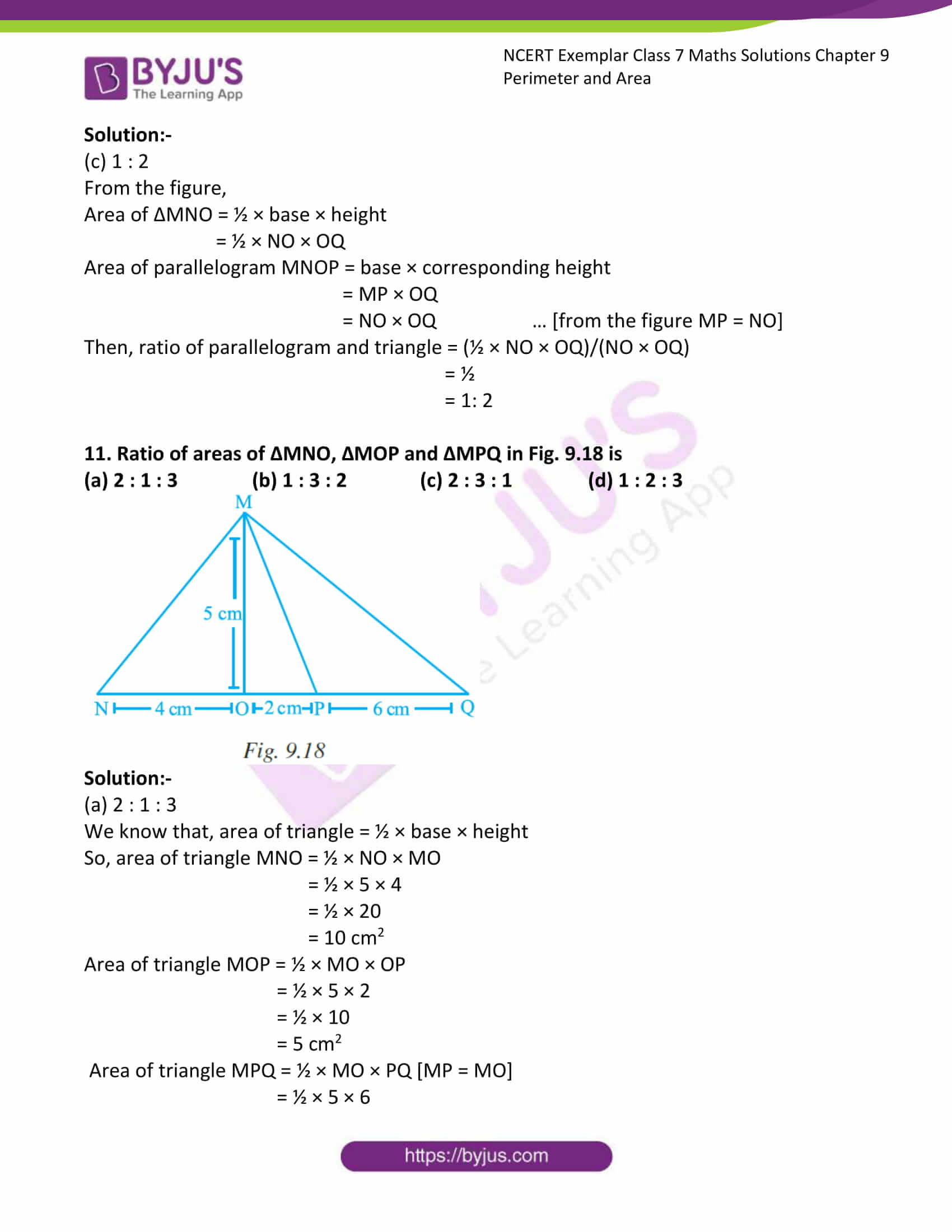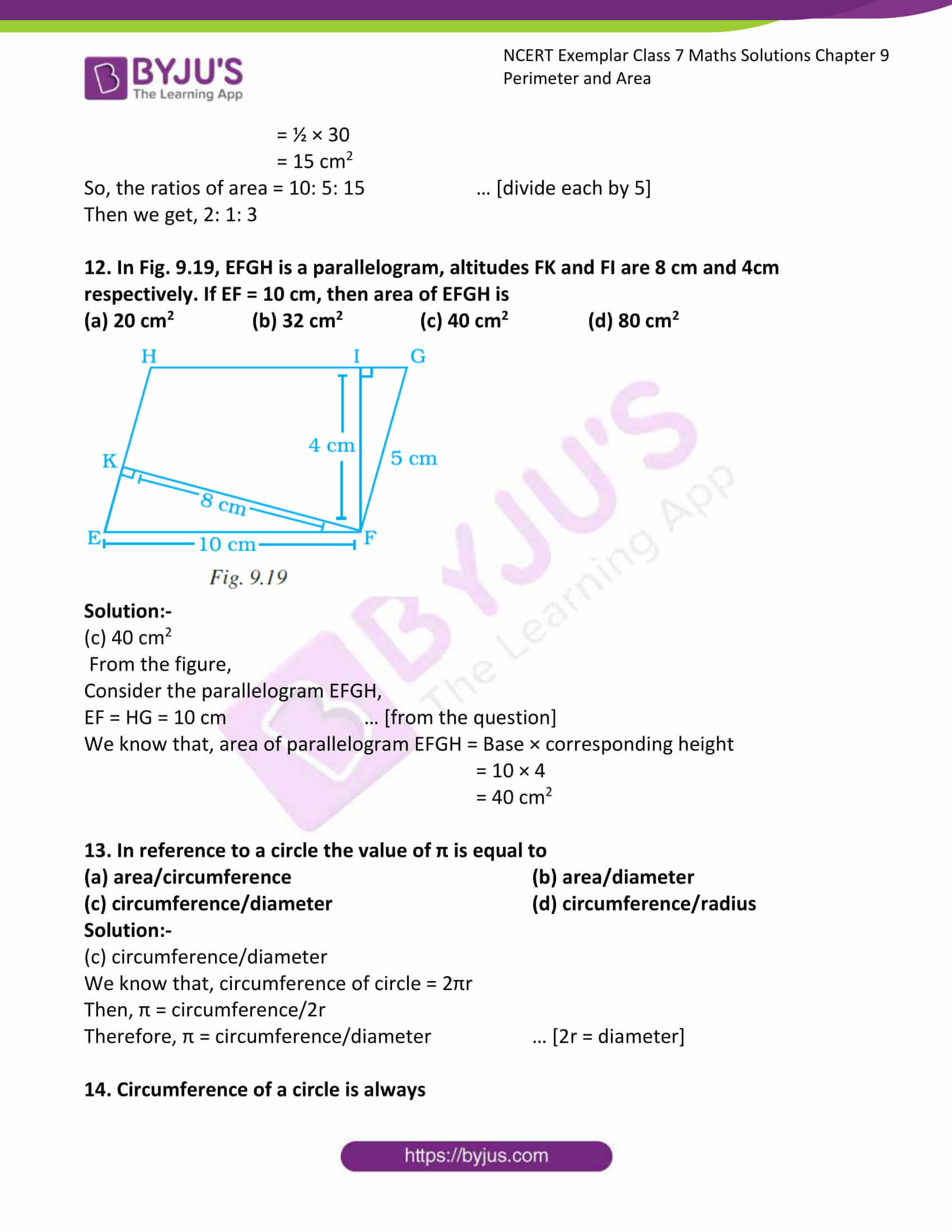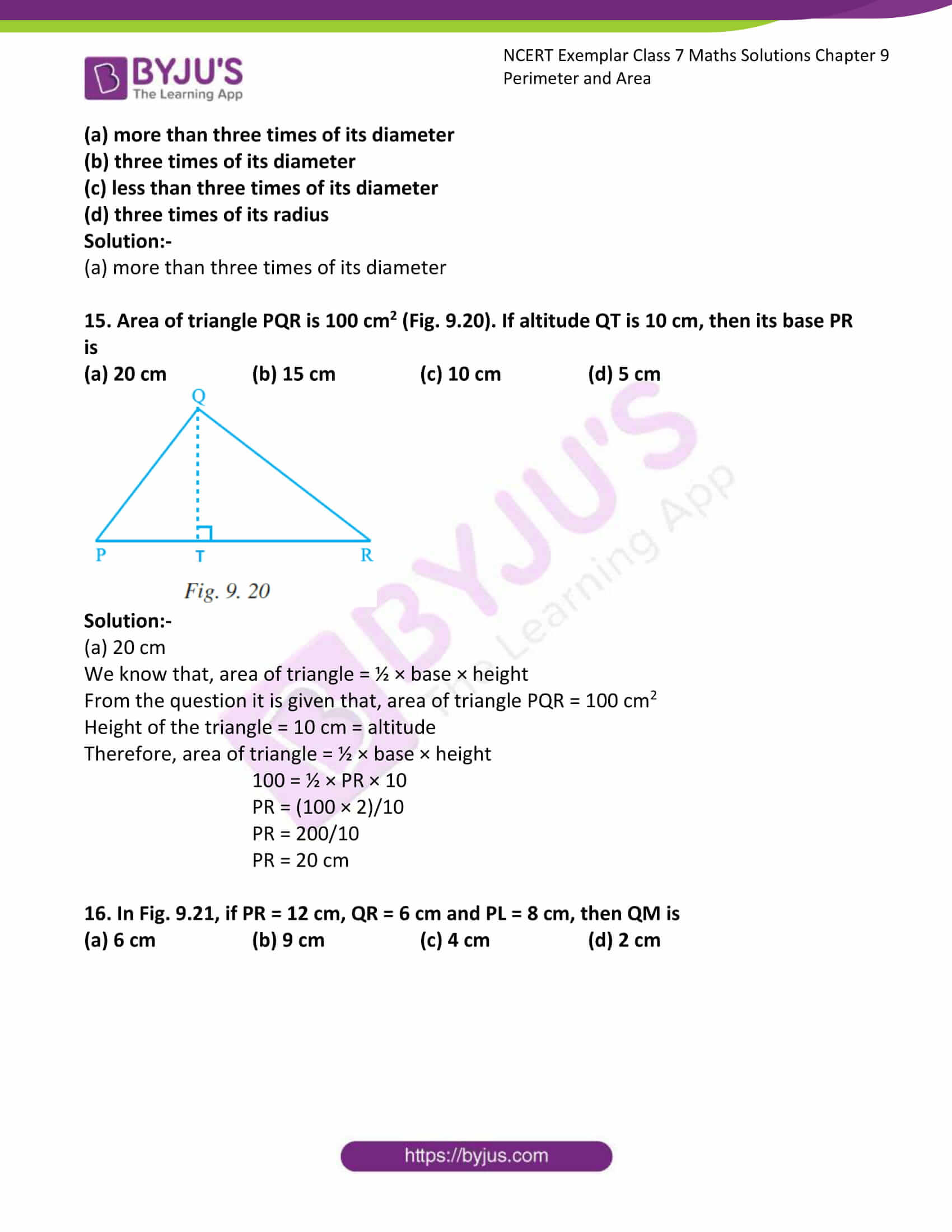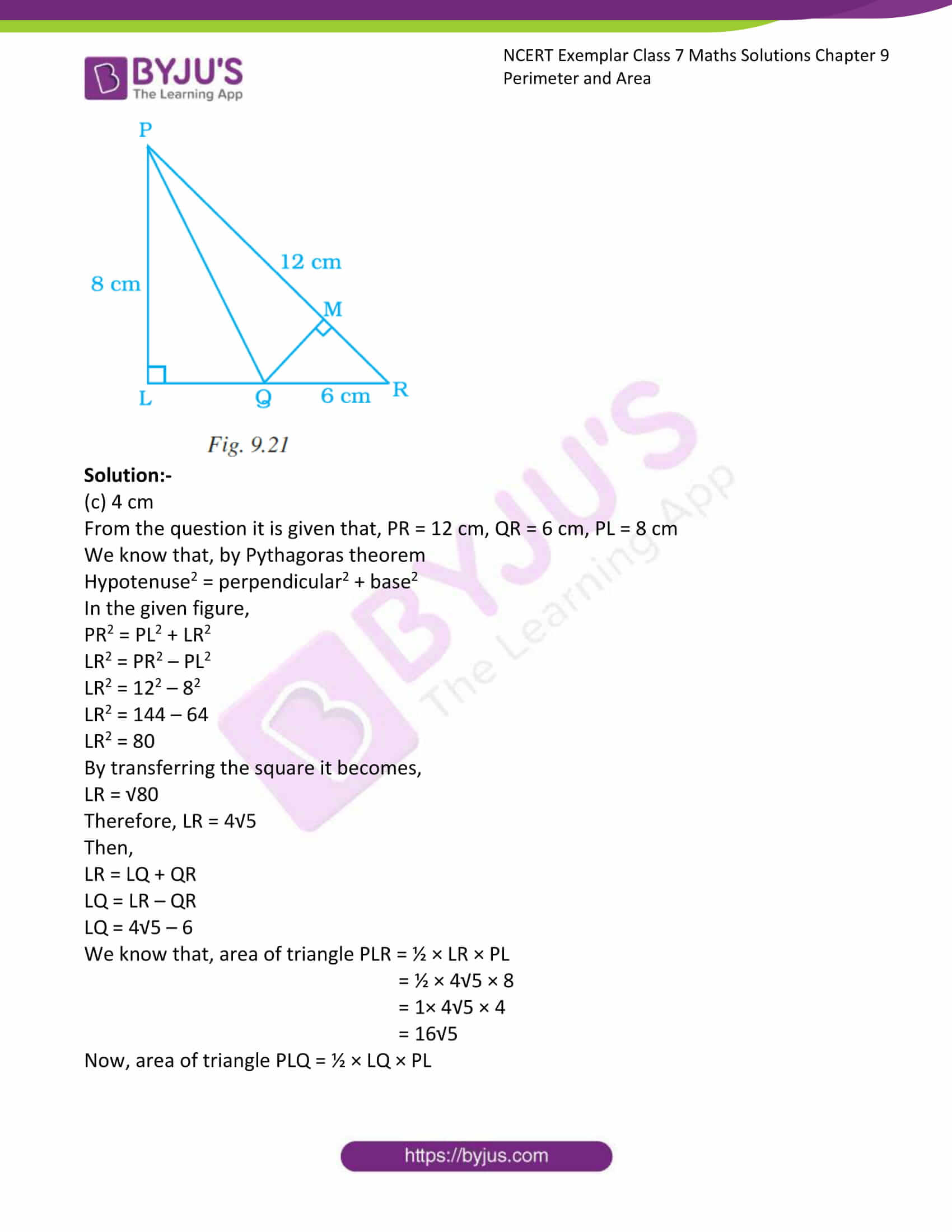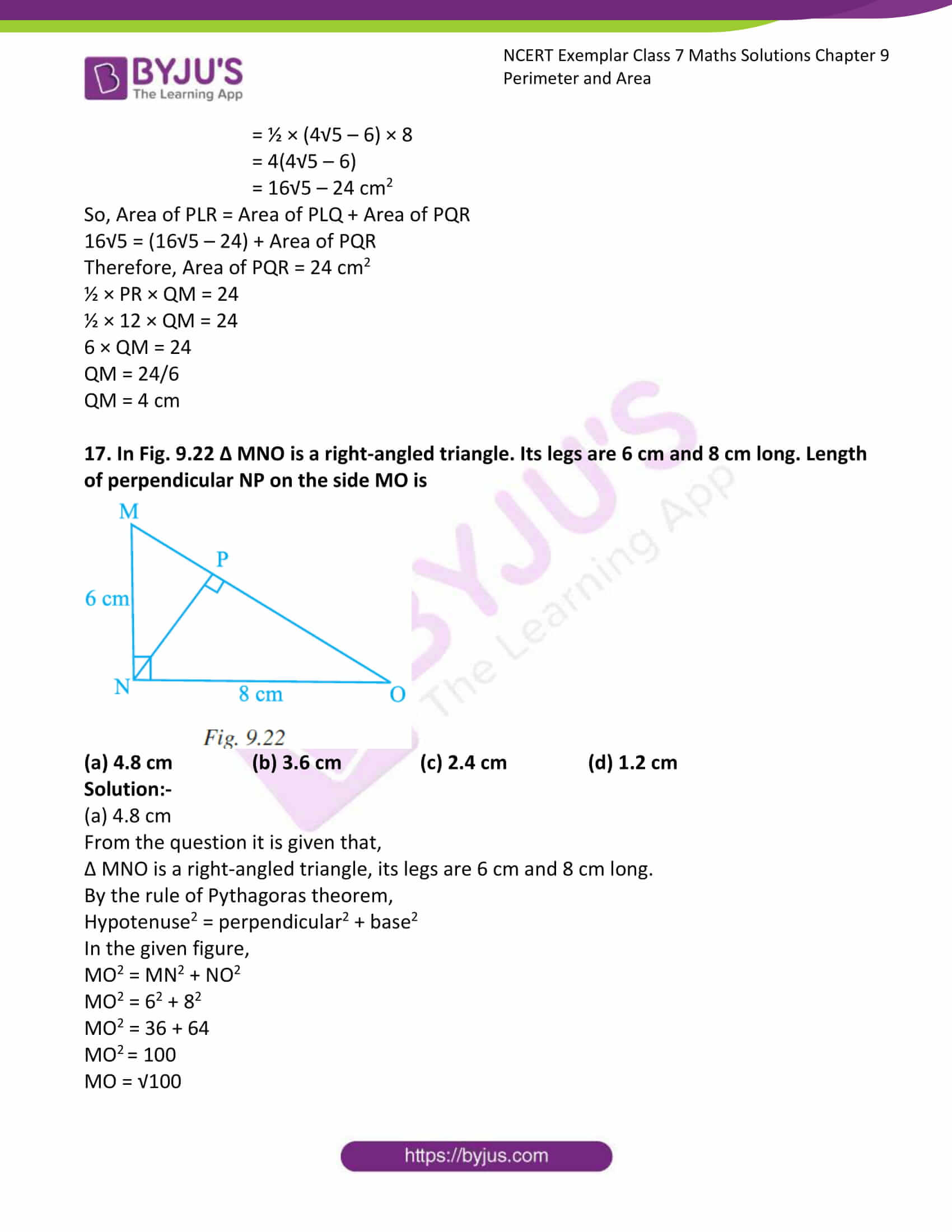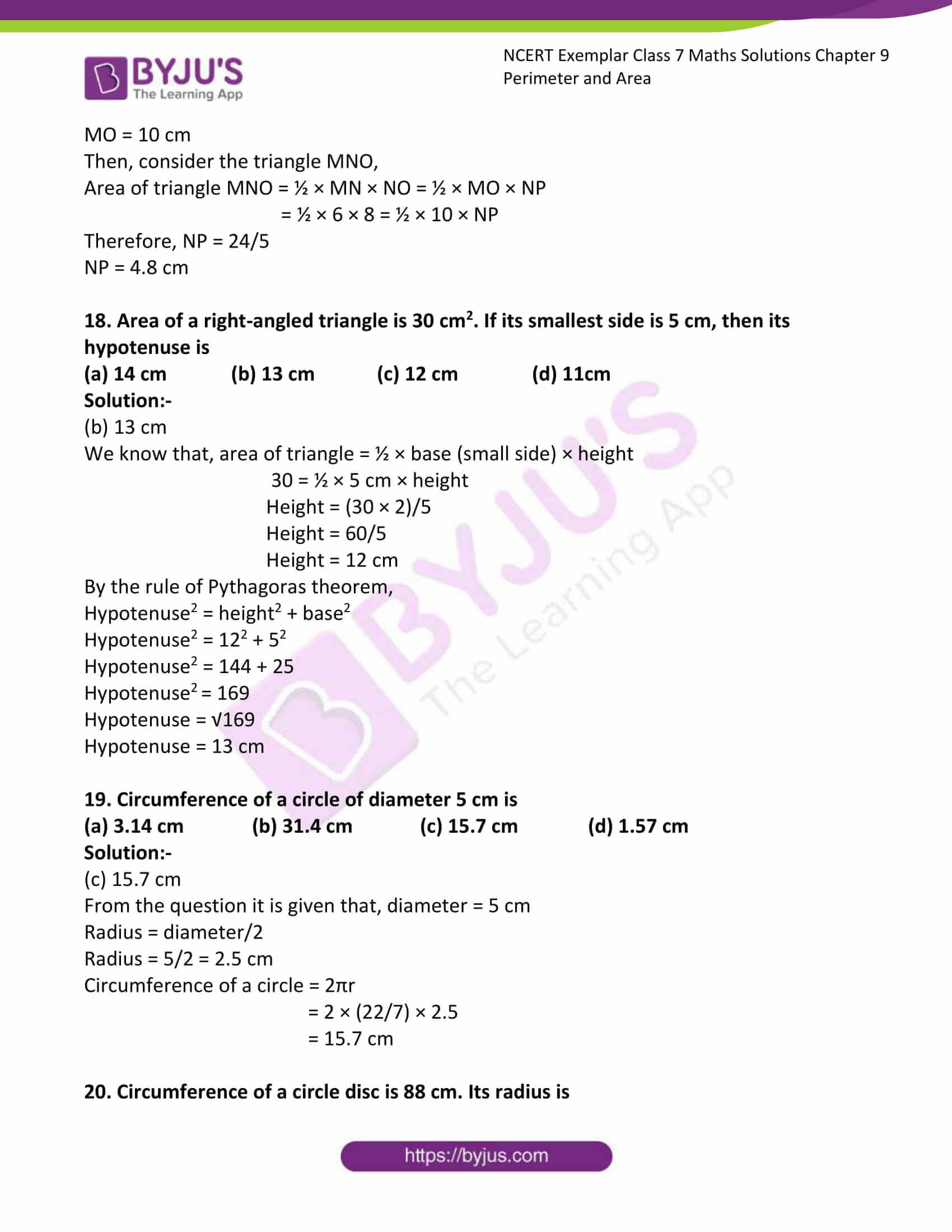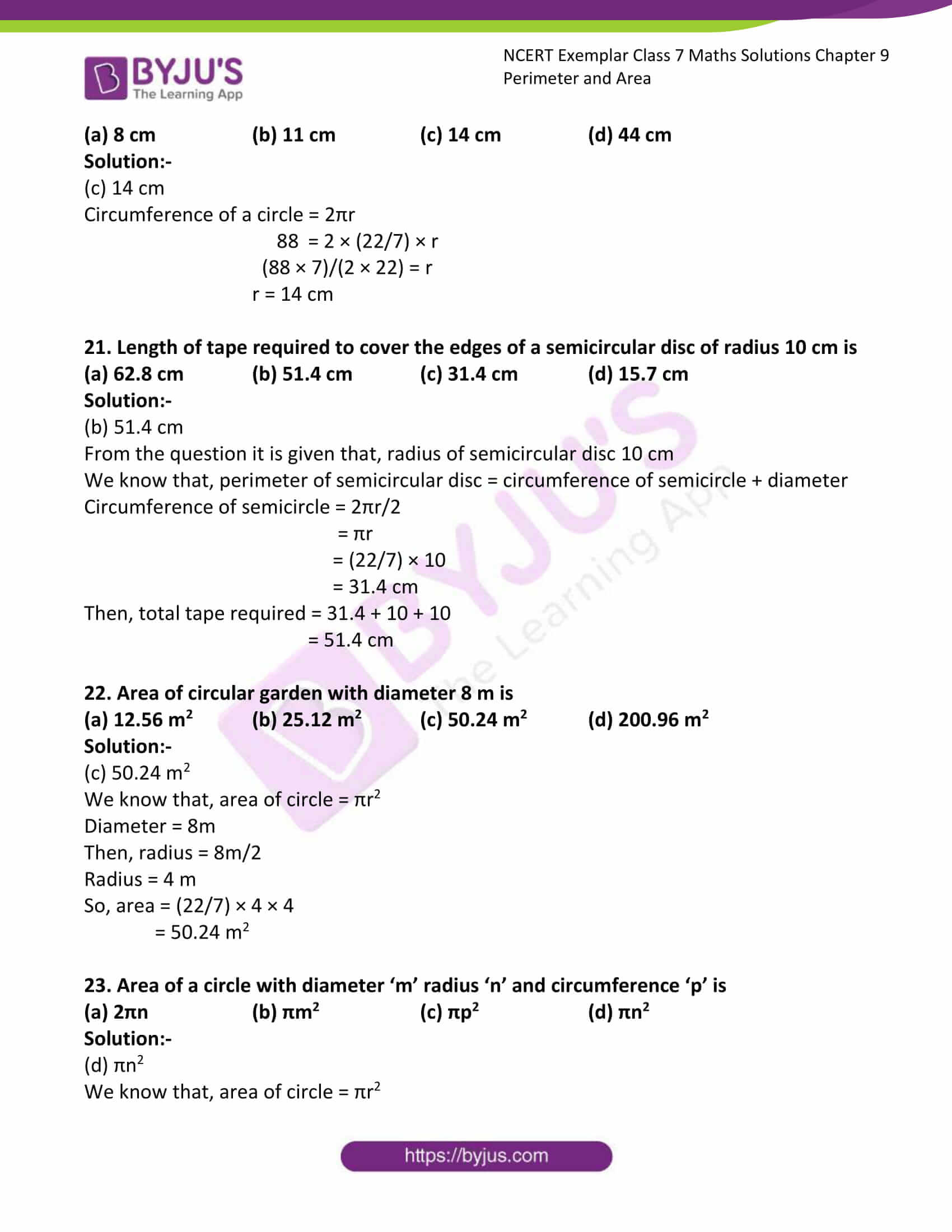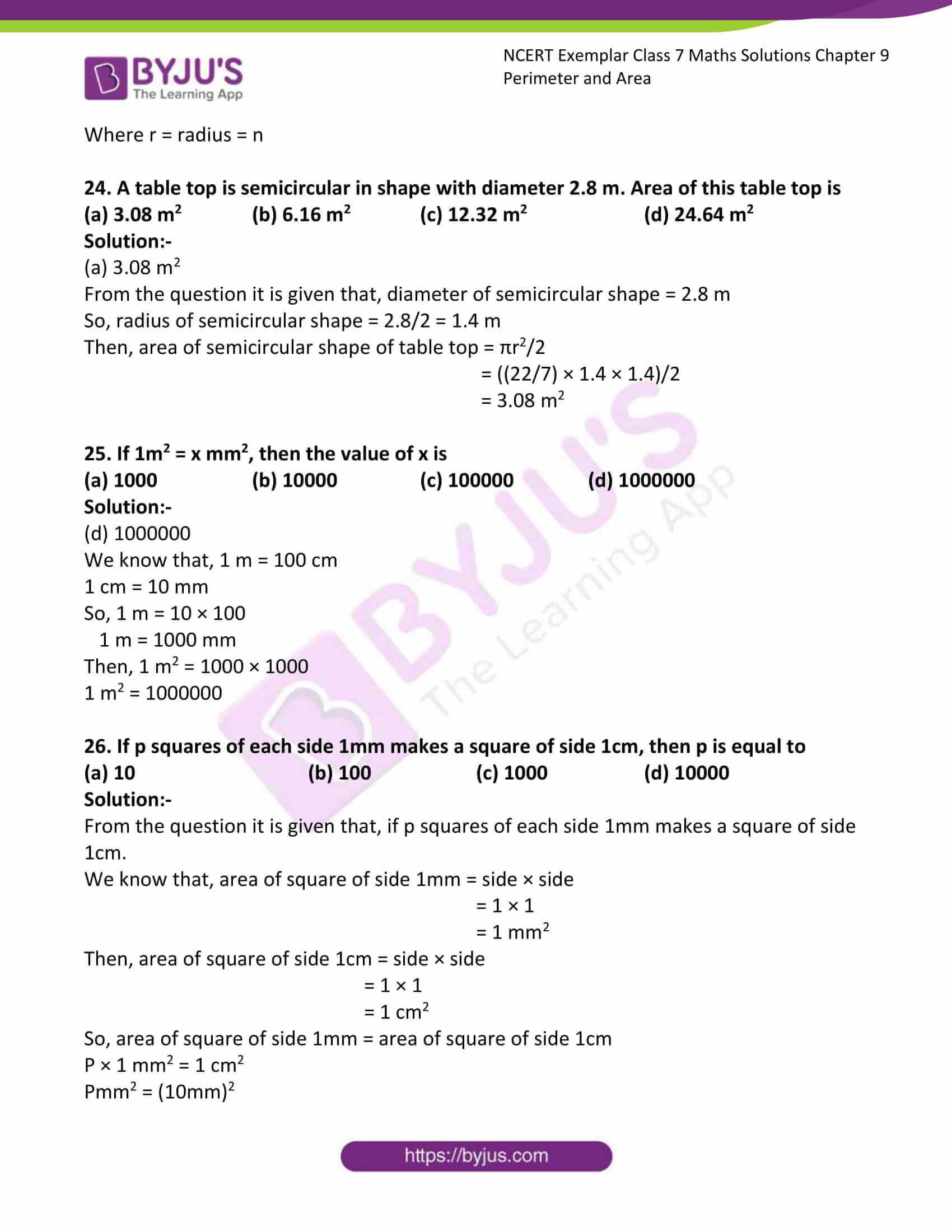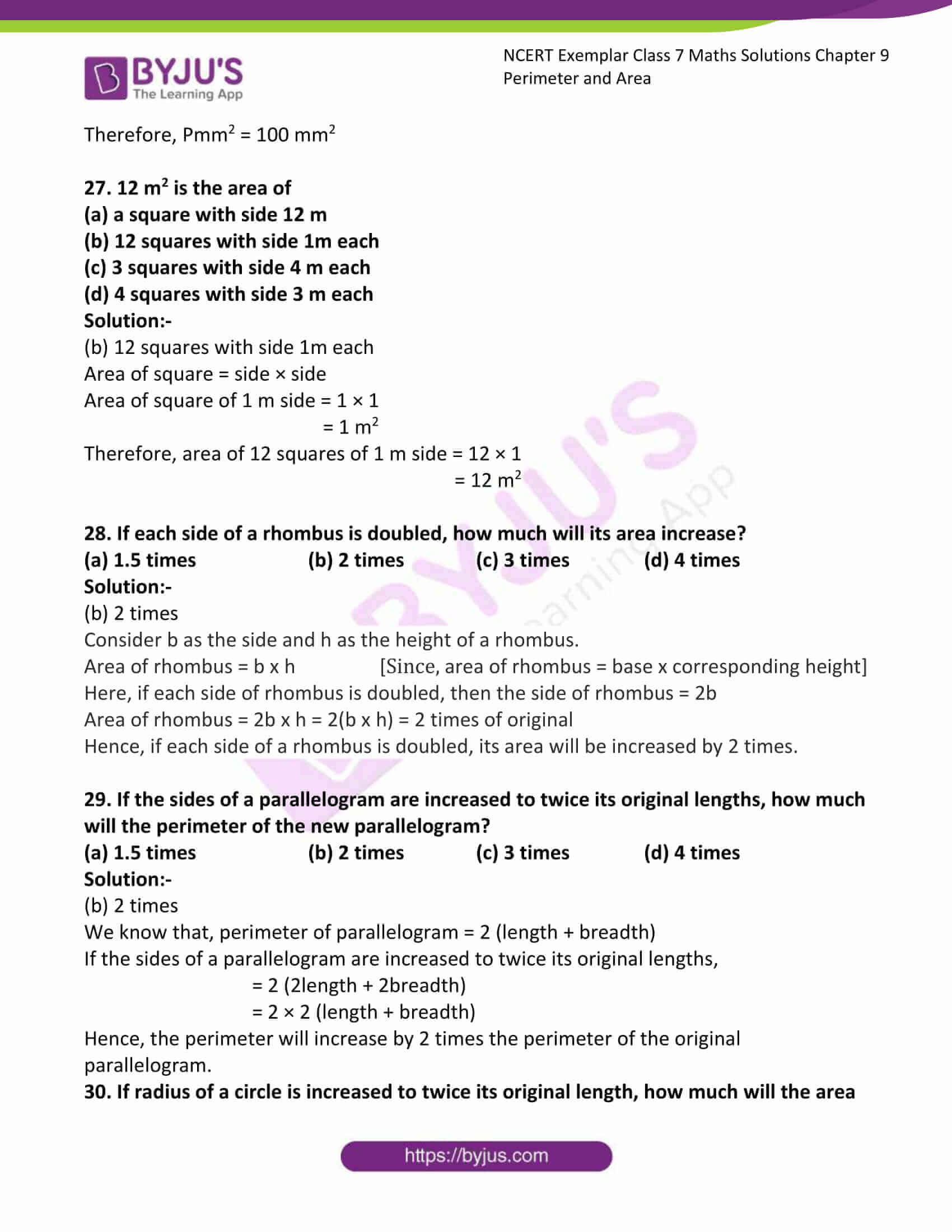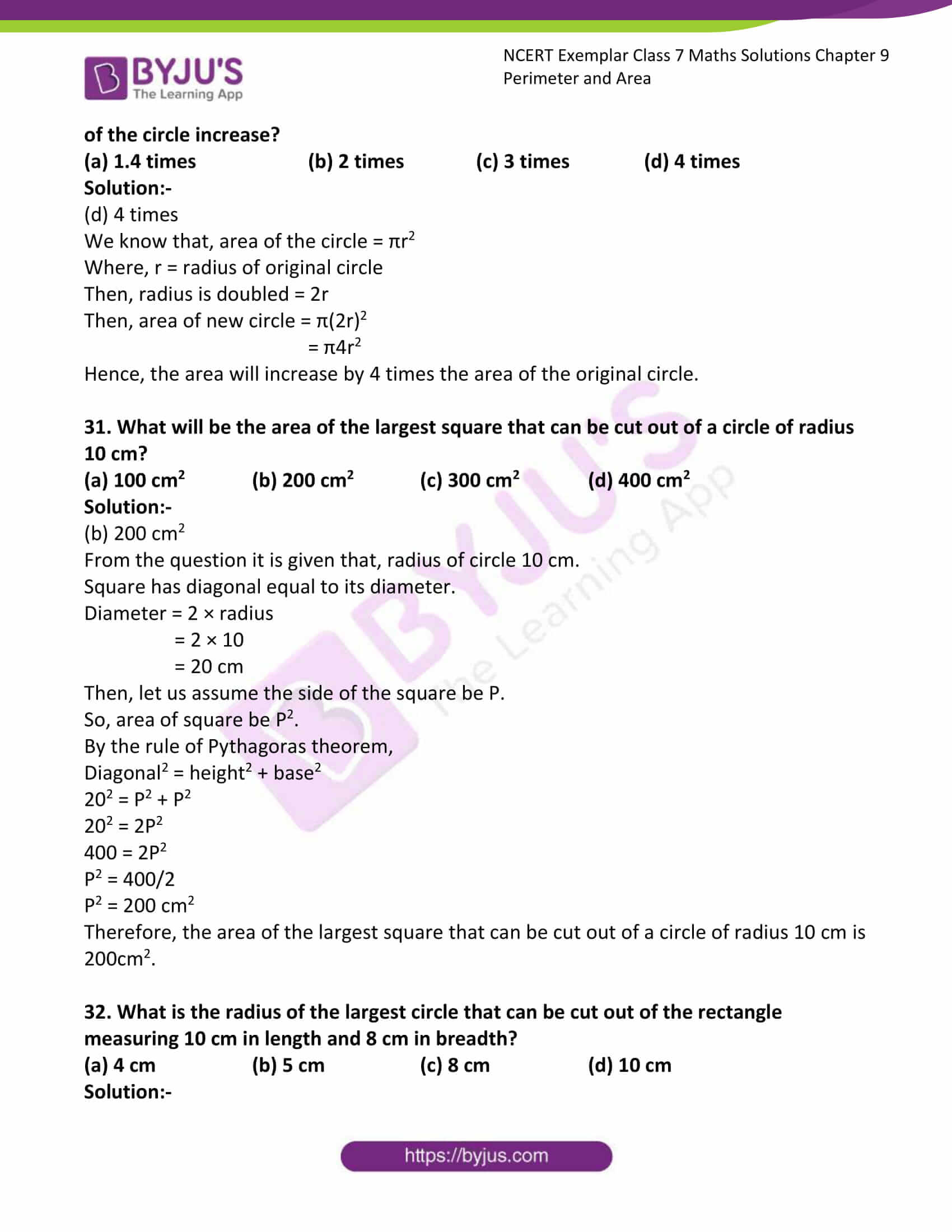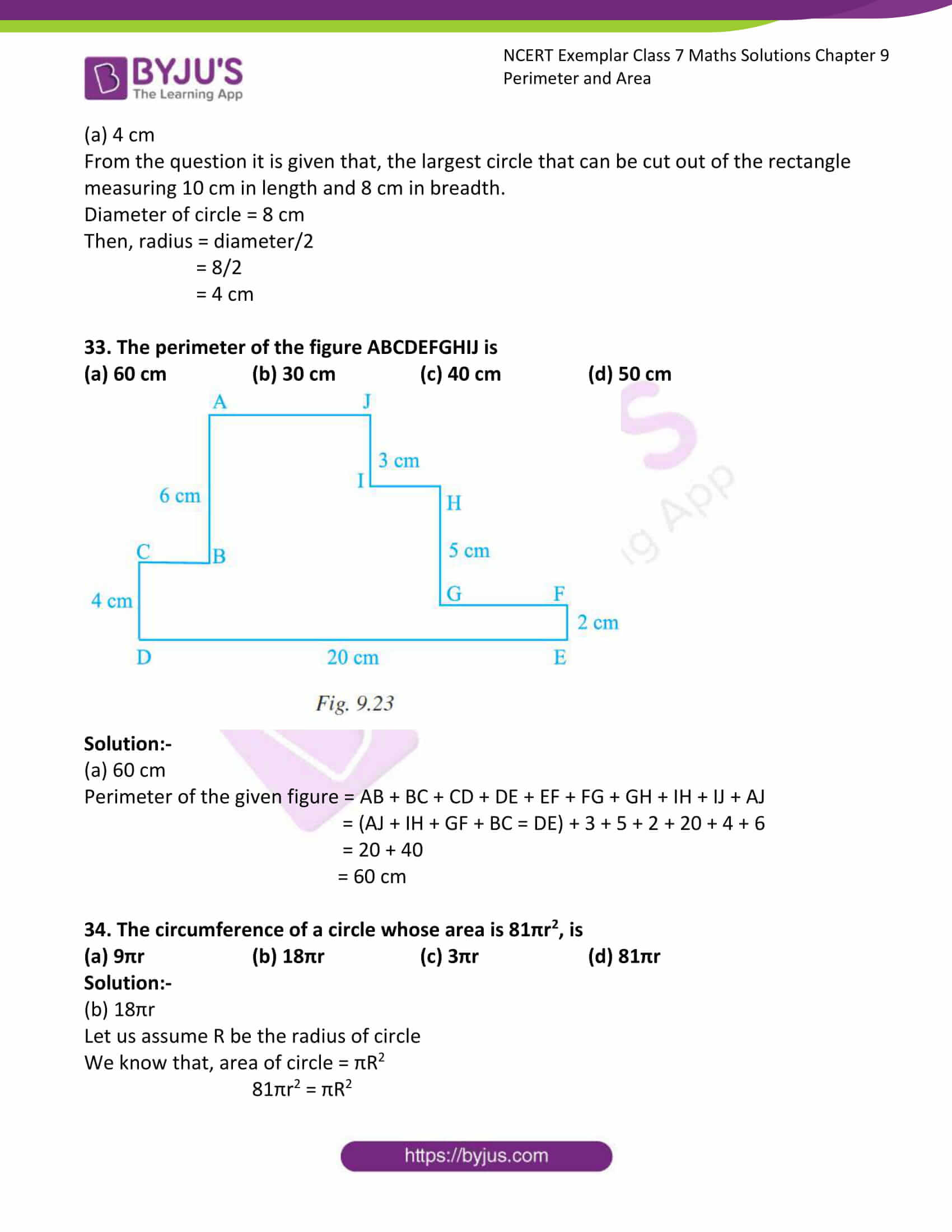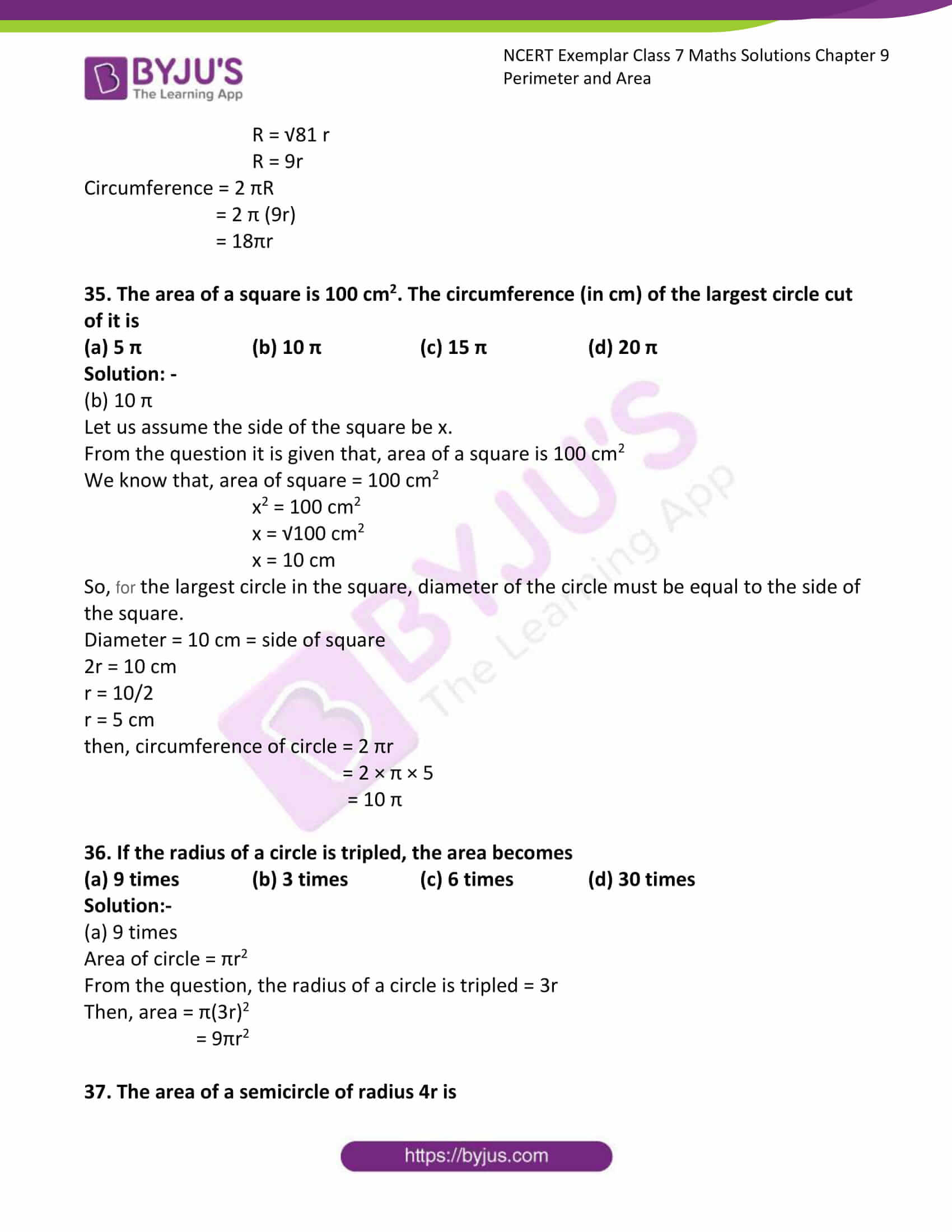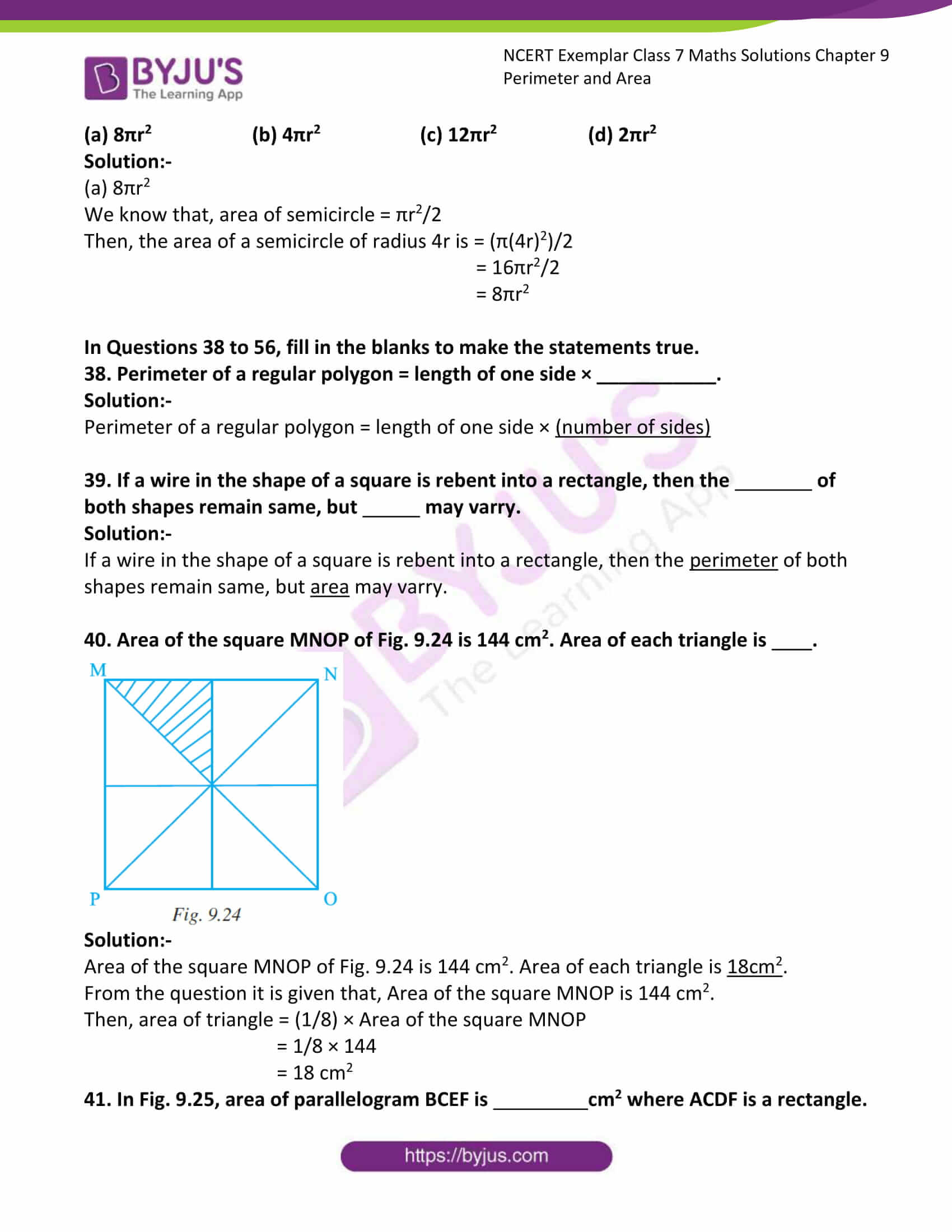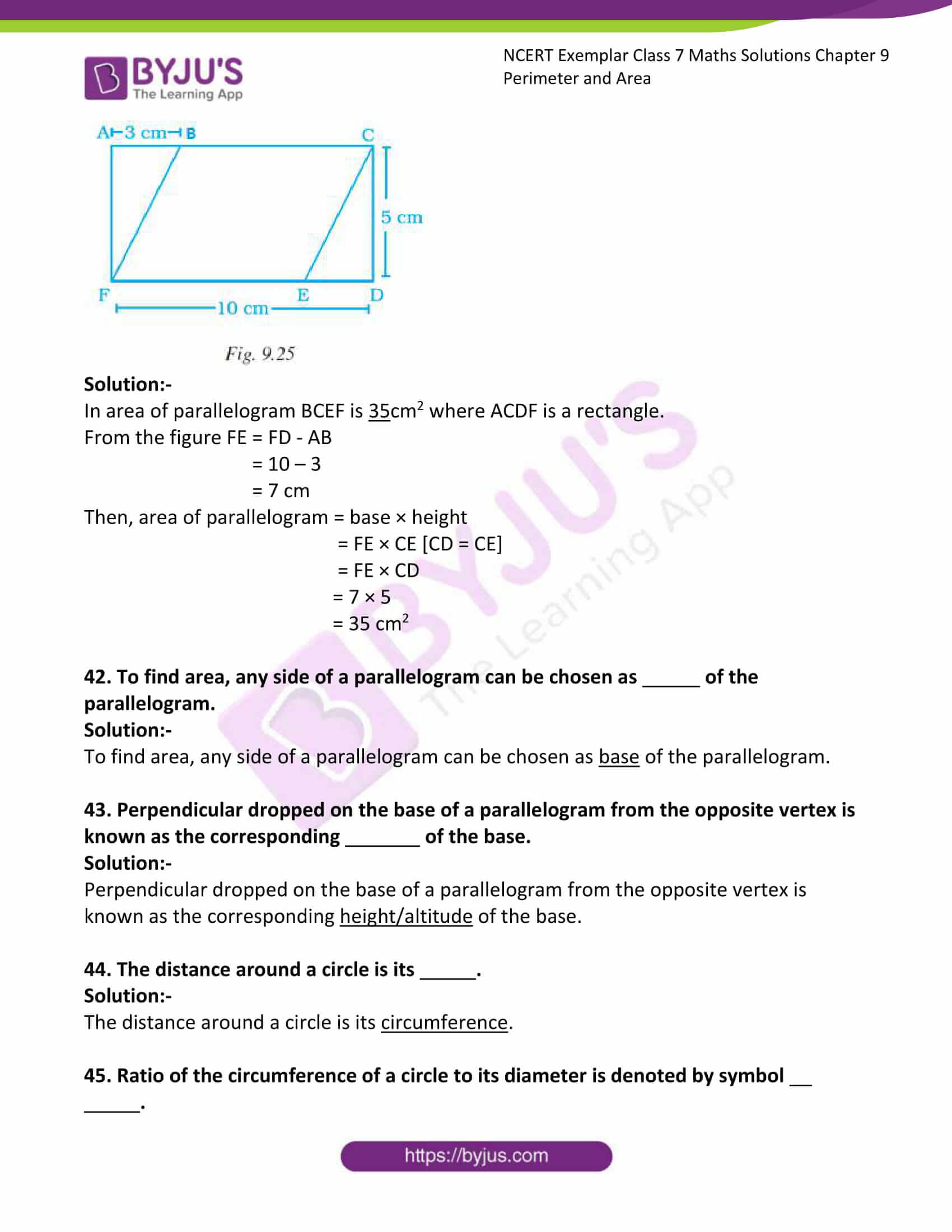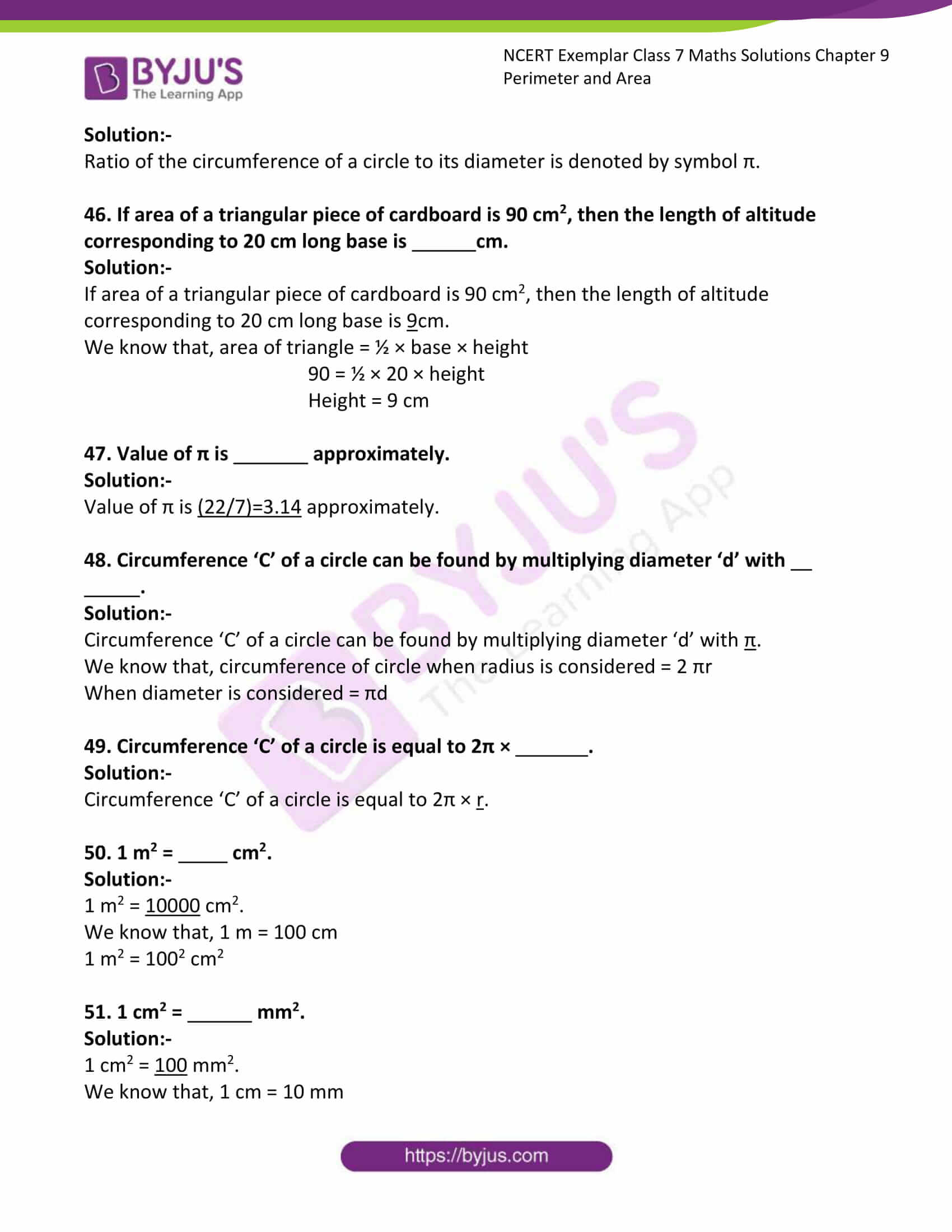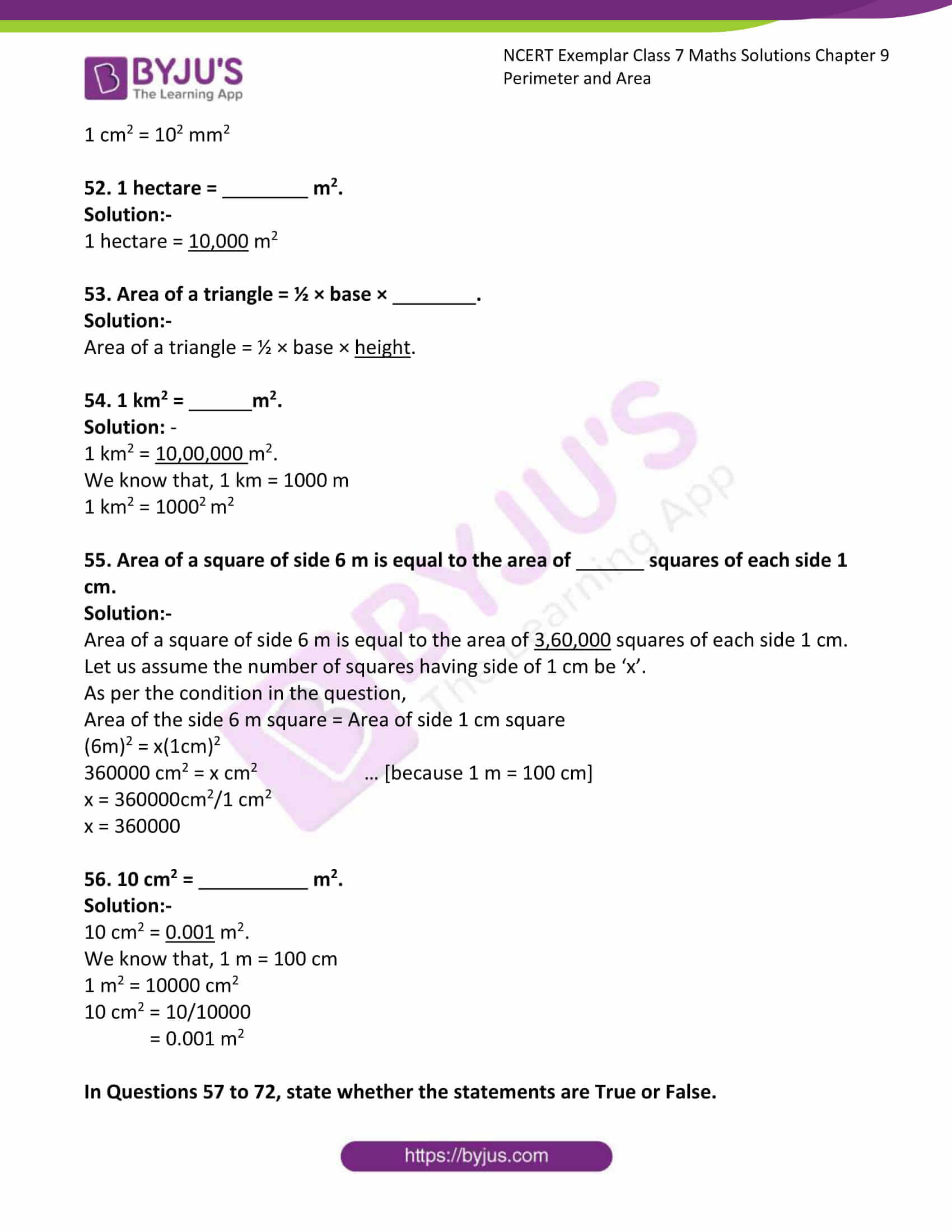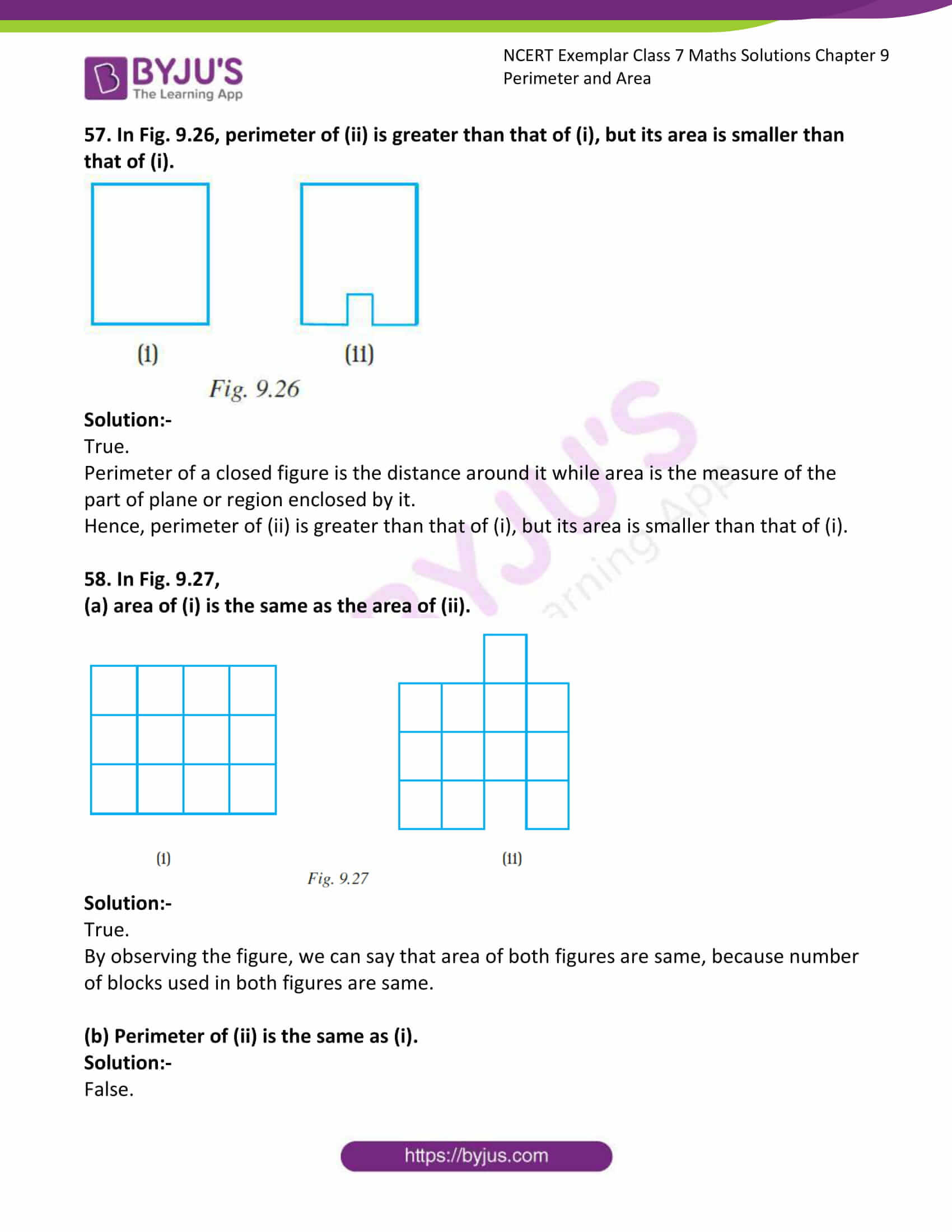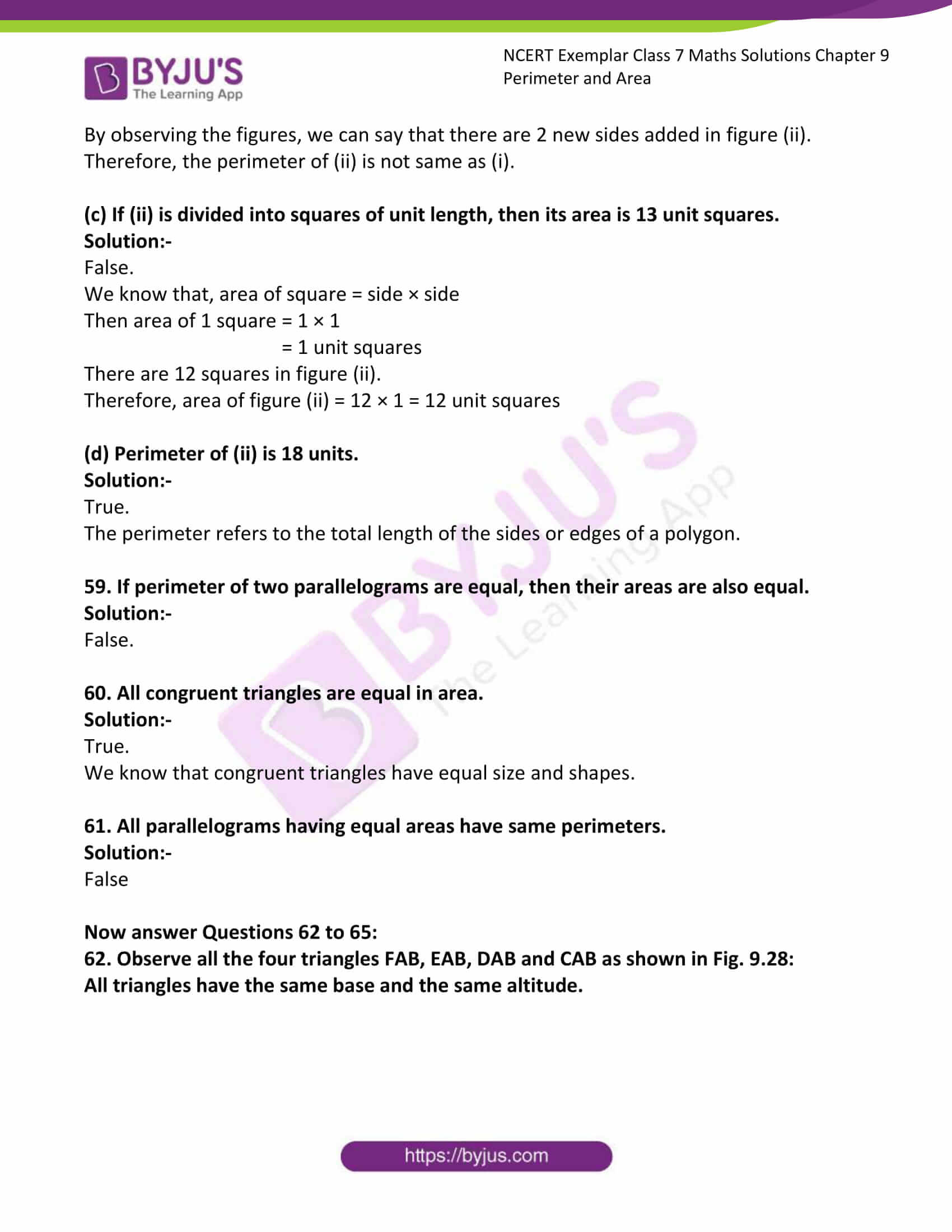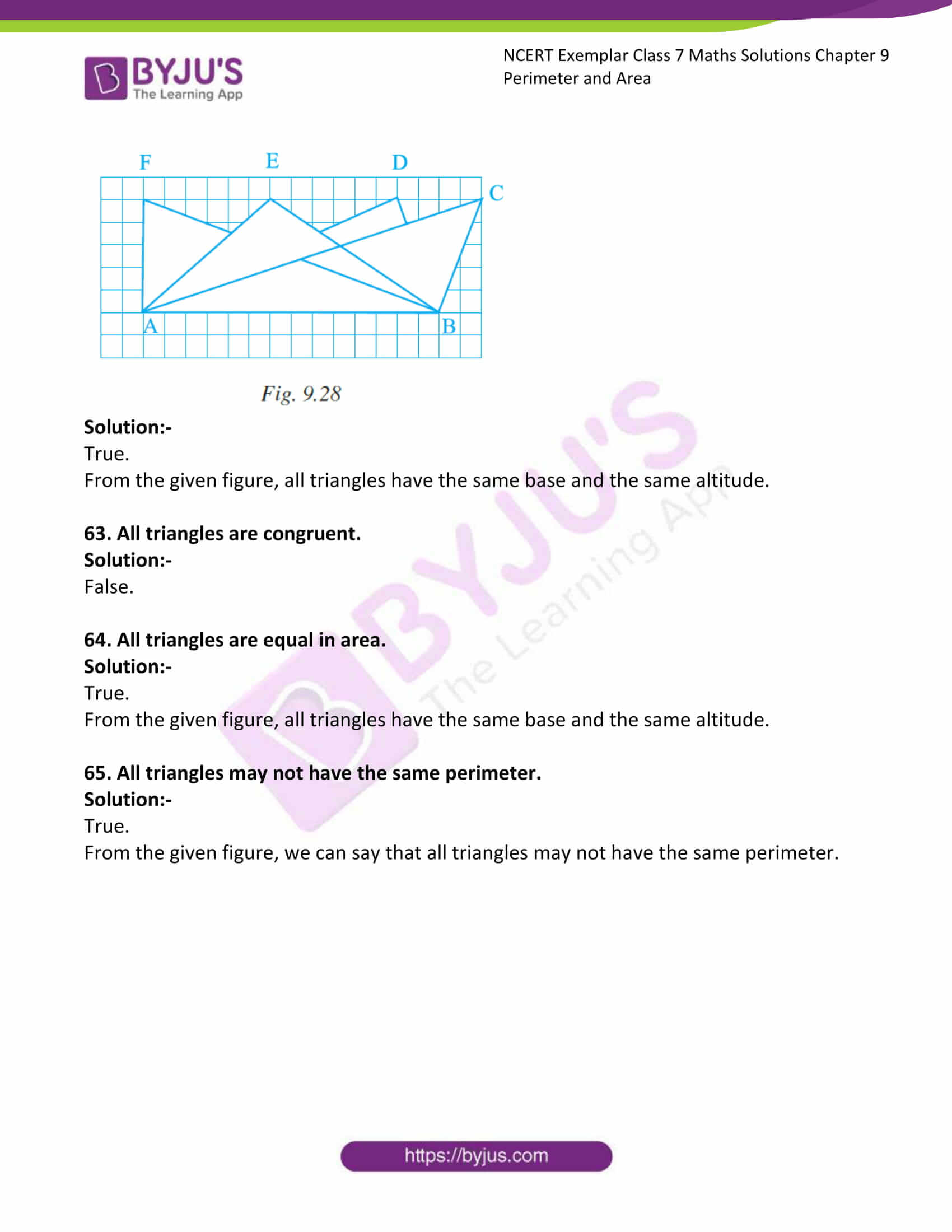### Access answers to Maths NCERT Exemplar Solutions for Class 7 Chapter 9 Perimeter and Area

Exercise Page: 266

In the Questions 1 to 37, there are four options, out of which one is correct. Choose the correct one.

1. Observe the shapes 1, 2, 3 and 4 in the figures. Which of the following statements is not correct?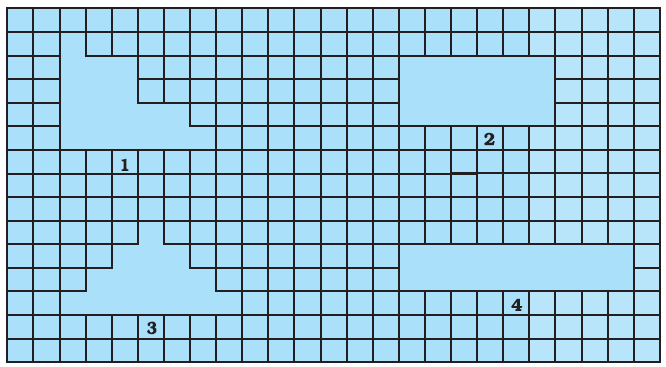(a) Shapes 1, 3 and 4 have different areas and different perimeters.

(b) Shapes 1 and 4 have the same area as well as the same perimeter.

(c) Shapes 1, 2 and 4 have the same area.

(d) Shapes 1, 3 and 4 have the same perimeter.

Solution:-

(a) Shapes 1, 3 and 4 have different areas and different perimeters.

Shapes 1, 3 and 4 have same area and same perimeter.

2. A rectangular piece of dimensions 3 cm × 2 cm was cut from a rectangular sheet of paper of dimensions 6 cm × 5 cm (Fig. 9.14).

Area of remaining sheet of paper is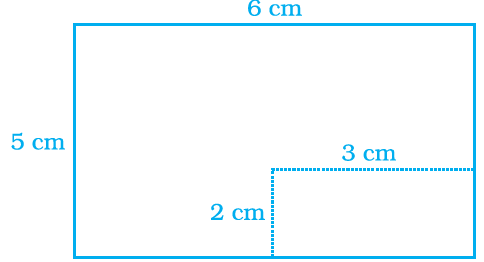(a) 30 cm2 (b) 36 cm2 (c) 24 cm2 (d) 22 cm2

Solution:-

(c) 24 cm2

First we have to calculate the area of rectangular piece = length × breadth

= 2 cm × 3 cm

= 6 cm2

So, area of sheet of paper of dimensions 6 cm × 5 cm

= 30 cm2

Then, Area of remaining sheet of paper is = 30 cm – 6 cm

= 24 cm2

3. 36 unit squares are joined to form a rectangle with the least perimeter. Perimeter of the rectangle is

(a) 12 units (b) 26 units (c) 24 units (d) 36 units

Solution:-

(b) 26 units

From the question it is given that, area of rectangle = 36 units2

36 can be written as = 6 × 6

= (2 × 3) × (2 × 3)

= 22 × 32

= 4 × 9

Then, the sides of the rectangle are 4 cm and 9 cm.

We know that, perimeter of the rectangle = 2 (length + breadth)

= 2 (4 + 9)

= 2 (13)

= 26 units

4. A wire is bent to form a square of side 22 cm. If the wire is rebent to form a circle, its radius is

(a) 22 cm (b) 14 cm (c) 11 cm (d) 7 cm

Solution:-

(b) 14 cm

From the question it is given that, side of square is 22 cm.

And also, perimeter of a square and circumference of circle are equal, because the length of the wire is same.

Perimeter of square = circumference of circle

4 × side = 2 × π × r

4 × 22 = 2 × (22/7) × r

r = (4 × 22 × 7)/(2 × 22)

r = 14 cm

Therefore, radius of circle is 14 cm.

5. Area of the circle obtained in Question 4 is

(a) 196 cm2 (b) 212 cm2 (c) 616 cm2 (d) 644 cm2

Solution:-

(c) 616 cm2

We know that, area of circle = πr2

= (22/7) × 14 × 14

= 22 × 14 × 2

= 616 cm2

6. Area of a rectangle and the area of a circle are equal. If the dimensions of the rectangle are 14cm × 11 cm, then radius of the circle is

(a) 21 cm (b) 10.5 cm (c) 14 cm (d) 7 cm.

Solution:-

(d) 7 cm

From the question it is given that, dimensions of rectangle length = 14 cm, breadth = 11 cm

As area of rectangle = area of circle

14 × 11 = (22/7) × r2

r2 = (14 × 11 × 7)/22

r2 = 49

r = √49

r = 7 cm

7. Area of shaded portion in Fig. 9.15 is

(a) 25 cm2 (b) 15 cm2 (c) 14 cm2 (d) 10 cm2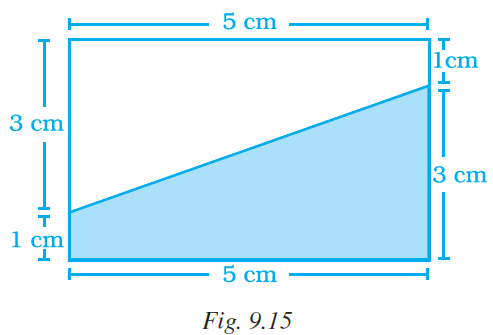Solution:-

(d) 10 cm2

From the figure, length of rectangle = 5 cm and,

breadth of the rectangle = 3 cm + 1 cm = 4 cm

So, area of rectangle = length × breadth

= 5 × 4

= 20 cm2

By observing the figure, the shaded part covered exactly half of the rectangle,

Therefore, area of shaded part is = area of rectangle/2

= 20/2

= 10 cm2

8. Area of parallelogram ABCD (Fig. 9.16) is not equal to

(a) DE × DC (b) BE × AD (c) BF × DC (d) BE × BC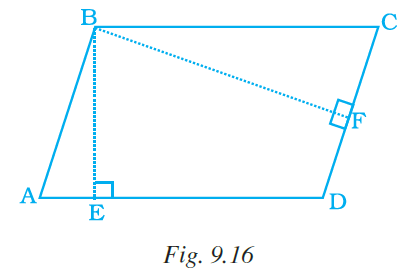Solution:-

(a) DE × DC

We know that, area of parallelogram = base × corresponding height

Then, area of parallelogram ABCD = DC × BF

AD × BE = BC × BE … [because AD = BC]

9. Area of triangle MNO of Fig. 9.17 is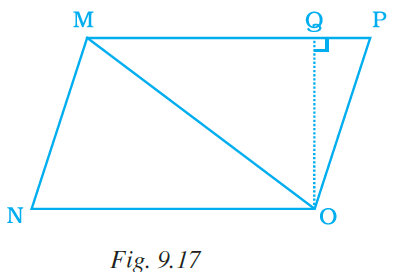(a) ½ MN × NO (b) ½ NO × MO

(c) ½ MN × OQ (d) ½ NO × OQ

Solution:-

(d) ½ NO × OQ

MNO is a triangle.

We know that, area of triangle = ½ (base × height)

= ½ × NO × OQ

10. Ratio of area of ∆MNO to the area of parallelogram MNOP in the same figure 9.17 is (a) 2 : 3 (b) 1 : 1 (c) 1 : 2 (d) 2 : 1Solution:-

(c) 1 : 2

From the figure,

Area of ∆MNO = ½ × base × height

= ½ × NO × OQ

Area of parallelogram MNOP = base × corresponding height

= MP × OQ

= NO × OQ … [from the figure MP = NO]

Then, ratio of parallelogram and triangle = (½ × NO × OQ)/(NO × OQ)

= ½

= 1: 2

11. Ratio of areas of ∆MNO, ∆MOP and ∆MPQ in Fig. 9.18 is

(a) 2 : 1 : 3 (b) 1 : 3 : 2 (c) 2 : 3 : 1 (d) 1 : 2 : 3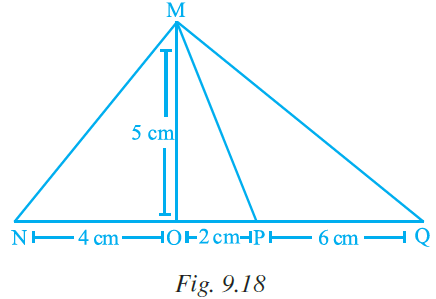Solution:-

(a) 2 : 1 : 3

We know that, area of triangle = ½ × base × height

So, area of triangle MNO = ½ × NO × MO

= ½ × 5 × 4

= ½ × 20

= 10 cm2

Area of triangle MOP = ½ × MO × OP

= ½ × 5 × 2

= ½ × 10

= 5 cm2

Area of triangle MPQ = ½ × MO × PQ [MP = MO]

= ½ × 5 × 6

= ½ × 30

= 15 cm2

So, the ratios of area = 10: 5: 15 … [divide each by 5]

Then we get, 2: 1: 3

12. In Fig. 9.19, EFGH is a parallelogram, altitudes FK and FI are 8 cm and 4cm respectively. If EF = 10 cm, then area of EFGH is

(a) 20 cm2 (b) 32 cm2 (c) 40 cm2 (d) 80 cm2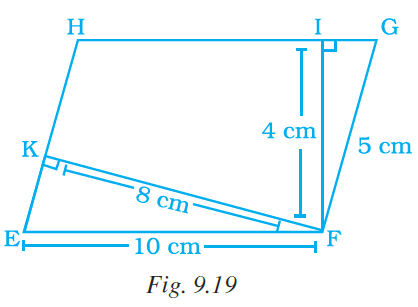Solution:-

(c) 40 cm2

From the figure,

Consider the parallelogram EFGH,

EF = HG = 10 cm … [from the question]

We know that, area of parallelogram EFGH = Base × corresponding height

= 10 × 4

= 40 cm2

13. In reference to a circle the value of π is equal to

(a) area/circumference (b) area/diameter

Solution:-

(c) circumference/diameter

We know that, circumference of circle = 2πr

Then, π = circumference/2r

Therefore, π = circumference/diameter … [2r = diameter]

14. Circumference of a circle is always

(a) more than three times of its diameter

(b) three times of its diameter

(c) less than three times of its diameter

(d) three times of its radius

Solution:-

(a) more than three times of its diameter

15. Area of triangle PQR is 100 cm2 (Fig. 9.20). If altitude QT is 10 cm, then its base PR is

(a) 20 cm (b) 15 cm (c) 10 cm (d) 5 cm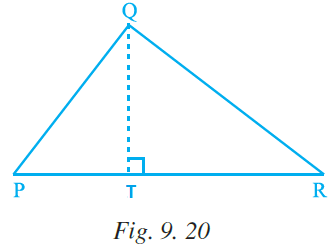Solution:-

(a) 20 cm

We know that, area of triangle = ½ × base × height

From the question it is given that, area of triangle PQR = 100 cm2

Height of the triangle = 10 cm = altitude

Therefore, area of triangle = ½ × base × height

100 = ½ × PR × 10

PR = (100 × 2)/10

PR = 200/10

PR = 20 cm

16. In Fig. 9.21, if PR = 12 cm, QR = 6 cm and PL = 8 cm, then QM is

(a) 6 cm (b) 9 cm (c) 4 cm (d) 2 cm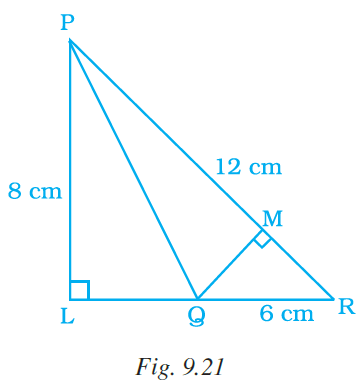Solution:-

(c) 4 cm

From the question it is given that, PR = 12 cm, QR = 6 cm, PL = 8 cm

We know that, by Pythagoras theorem

Hypotenuse2 = perpendicular2 + base2

In the given figure,

PR2 = PL2 + LR2

LR2 = PR2 – PL2

LR2 = 122 – 82

LR2 = 144 – 64

LR2 = 80

By transferring the square it becomes,

LR = √80

Therefore, LR = 4√5

Then,

LR = LQ + QR

LQ = LR – QR

LQ = 4√5 – 6

We know that, area of triangle PLR = ½ × LR × PL

= ½ × 4√5 × 8

= 1× 4√5 × 4

= 16√5

Now, area of triangle PLQ = ½ × LQ × PL

= ½ × (4√5 – 6) × 8

= 4(4√5 – 6)

= 16√5 – 24 cm2

So, Area of PLR = Area of PLQ + Area of PQR

16√5 = (16√5 – 24) + Area of PQR

Therefore, Area of PQR = 24 cm2

½ × PR × QM = 24

½ × 12 × QM = 24

6 × QM = 24

QM = 24/6

QM = 4 cm

17. In Fig. 9.22 ∆ MNO is a right-angled triangle. Its legs are 6 cm and 8 cm long. Length of perpendicular NP on the side MO is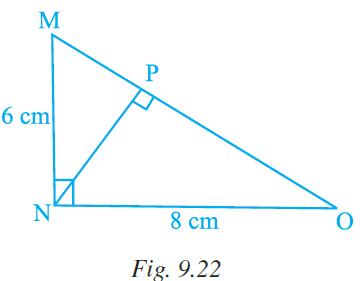(a) 4.8 cm (b) 3.6 cm (c) 2.4 cm (d) 1.2 cm

Solution:-

(a) 4.8 cm

From the question it is given that,

∆ MNO is a right-angled triangle, its legs are 6 cm and 8 cm long.

By the rule of Pythagoras theorem,

Hypotenuse2 = perpendicular2 + base2

In the given figure,

MO2 = MN2 + NO2

MO2 = 62 + 82

MO2 = 36 + 64

MO2 = 100

MO = √100

MO = 10 cm

Then, consider the triangle MNO,

Area of triangle MNO = ½ × MN × NO = ½ × MO × NP

= ½ × 6 × 8 = ½ × 10 × NP

Therefore, NP = 24/5

NP = 4.8 cm

18. Area of a right-angled triangle is 30 cm2. If its smallest side is 5 cm, then its hypotenuse is

(a) 14 cm (b) 13 cm (c) 12 cm (d) 11cm

Solution:-

(b) 13 cm

We know that, area of triangle = ½ × base (small side) × height

30 = ½ × 5 cm × height

Height = (30 × 2)/5

Height = 60/5

Height = 12 cm

By the rule of Pythagoras theorem,

Hypotenuse2 = height2 + base2

Hypotenuse2 = 122 + 52

Hypotenuse2 = 144 + 25

Hypotenuse2 = 169

Hypotenuse = √169

Hypotenuse = 13 cm

19. Circumference of a circle of diameter 5 cm is

(a) 3.14 cm (b) 31.4 cm (c) 15.7 cm (d) 1.57 cm

Solution:-

(c) 15.7 cm

From the question it is given that, diameter = 5 cm

Radius = 5/2 = 2.5 cm

Circumference of a circle = 2πr

= 2 × (22/7) × 2.5

= 15.7 cm

20. Circumference of a circle disc is 88 cm. Its radius is

(a) 8 cm (b) 11 cm (c) 14 cm (d) 44 cm

Solution:-

(c) 14 cm

Circumference of a circle = 2πr

88 = 2 × (22/7) × r

(88 × 7)/(2 × 22) = r

r = 14 cm

21. Length of tape required to cover the edges of a semicircular disc of radius 10 cm is (a) 62.8 cm (b) 51.4 cm (c) 31.4 cm (d) 15.7 cm

Solution:-

(b) 51.4 cm

From the question it is given that, radius of semicircular disc 10 cm

We know that, perimeter of semicircular disc = circumference of semicircle + diameter

Circumference of semicircle = 2πr/2

= πr

= (22/7) × 10

= 31.4 cm

Then, total tape required = 31.4 + 10 + 10

= 51.4 cm

22. Area of circular garden with diameter 8 m is

(a) 12.56 m2 (b) 25.12 m2 (c) 50.24 m2 (d) 200.96 m2

Solution:-

(c) 50.24 m2

We know that, area of circle = πr2

Diameter = 8m

So, area = (22/7) × 4 × 4

= 50.24 m2

23. Area of a circle with diameter ‘m’ radius ‘n’ and circumference ‘p’ is

(a) 2πn (b) πm2 (c) πp2 (d) πn2

Solution:-

(d) πn2

We know that, area of circle = πr2

Where r = radius = n

24. A table top is semicircular in shape with diameter 2.8 m. Area of this table top is

(a) 3.08 m2 (b) 6.16 m2 (c) 12.32 m2 (d) 24.64 m2

Solution:-

(a) 3.08 m2

From the question it is given that, diameter of semicircular shape = 2.8 m

So, radius of semicircular shape = 2.8/2 = 1.4 m

Then, area of semicircular shape of table top = πr2/2

= ((22/7) × 1.4 × 1.4)/2

= 3.08 m2

25. If 1m2 = x mm2, then the value of x is

(a) 1000 (b) 10000 (c) 100000 (d) 1000000

Solution:-

(d) 1000000

We know that, 1 m = 100 cm

1 cm = 10 mm

So, 1 m = 10 × 100

1 m = 1000 mm

Then, 1 m2 = 1000 × 1000

1 m2 = 1000000

26. If p squares of each side 1mm makes a square of side 1cm, then p is equal to

(a) 10 (b) 100 (c) 1000 (d) 10000

Solution:-

From the question it is given that, if p squares of each side 1mm makes a square of side 1cm.

We know that, area of square of side 1mm = side × side

= 1 × 1

= 1 mm2

Then, area of square of side 1cm = side × side

= 1 × 1

= 1 cm2

So, area of square of side 1mm = area of square of side 1cm

P × 1 mm2 = 1 cm2

Pmm2 = (10mm)2

Therefore, Pmm2 = 100 mm2

27. 12 m2 is the area of

(a) a square with side 12 m

(b) 12 squares with side 1m each

(c) 3 squares with side 4 m each

(d) 4 squares with side 3 m each

Solution:-

(b) 12 squares with side 1m each

Area of square = side × side

Area of square of 1 m side = 1 × 1

= 1 m2

Therefore, area of 12 squares of 1 m side = 12 × 1

= 12 m2

28. If each side of a rhombus is doubled, how much will its area increase?

(a) 1.5 times (b) 2 times (c) 3 times (d) 4 times

Solution:-

(b) 2 times

Consider b as the side and h as the height of a rhombus.

Area of rhombus = b x h                 [Since, area of rhombus = base x corresponding height]
Here, if each side of rhombus is doubled, then the side of rhombus = 2b

Area of rhombus = 2b x h = 2(b x h) = 2 times of original

Hence, if each side of a rhombus is doubled, its area will be increased by 2 times.

29. If the sides of a parallelogram are increased to twice its original lengths, how much will the perimeter of the new parallelogram?

(a) 1.5 times (b) 2 times (c) 3 times (d) 4 times

Solution:-

(b) 2 times

We know that, perimeter of parallelogram = 2 (length + breadth)

If the sides of a parallelogram are increased to twice its original lengths,

= 2 × 2 (length + breadth)

Hence, the perimeter will increase by 2 times the perimeter of the original parallelogram.

30. If radius of a circle is increased to twice its original length, how much will the area of the circle increase?

(a) 1.4 times (b) 2 times (c) 3 times (d) 4 times

Solution:-

(d) 4 times

We know that, area of the circle = πr2

Where, r = radius of original circle

Then, radius is doubled = 2r

Then, area of new circle = π(2r)2

= π4r2

Hence, the area will increase by 4 times the area of the original circle.

31. What will be the area of the largest square that can be cut out of a circle of radius 10 cm?

(a) 100 cm2 (b) 200 cm2 (c) 300 cm2 (d) 400 cm2

Solution:-

(b) 200 cm2

From the question it is given that, radius of circle 10 cm.

Square has diagonal equal to its diameter.

= 2 × 10

= 20 cm

Then, let us assume the side of the square be P.

So, area of square be P2.

By the rule of Pythagoras theorem,

Diagonal2 = height2 + base2

202 = P2 + P2

202 = 2P2

400 = 2P2

P2 = 400/2

P2 = 200 cm2

Therefore, the area of the largest square that can be cut out of a circle of radius 10 cm is 200cm2.

32. What is the radius of the largest circle that can be cut out of the rectangle measuring 10 cm in length and 8 cm in breadth?

(a) 4 cm (b) 5 cm (c) 8 cm (d) 10 cm

Solution:-

(a) 4 cm

From the question it is given that, the largest circle that can be cut out of the rectangle measuring 10 cm in length and 8 cm in breadth.

Diameter of circle = 8 cm

= 8/2

= 4 cm

33. The perimeter of the figure ABCDEFGHIJ is

(a) 60 cm (b) 30 cm (c) 40 cm (d) 50 cm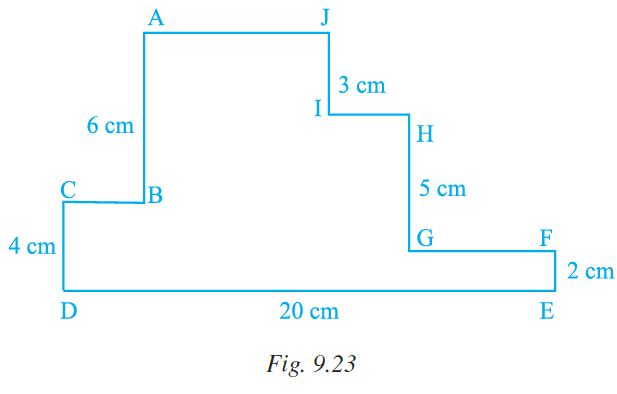Solution:-

(a) 60 cm

Perimeter of the given figure = AB + BC + CD + DE + EF + FG + GH + IH + IJ + AJ

= (AJ + IH + GF + BC = DE) + 3 + 5 + 2 + 20 + 4 + 6

= 20 + 40

= 60 cm

34. The circumference of a circle whose area is 81πr2, is

(a) 9πr (b) 18πr (c) 3πr (d) 81πr

Solution:-

(b) 18πr

Let us assume R be the radius of circle

We know that, area of circle = πR2

81πr2 = πR2

R = √81 r

R = 9r

Circumference = 2 πR

= 2 π (9r)

= 18πr

35. The area of a square is 100 cm2. The circumference (in cm) of the largest circle cut of it is

(a) 5 π (b) 10 π (c) 15 π (d) 20 π

Solution: –

(b) 10 π

Let us assume the side of the square be x.

From the question it is given that, area of a square is 100 cm2

We know that, area of square = 100 cm2

x2 = 100 cm2

x = √100 cm2

x = 10 cm

So, for the largest circle in the square, diameter of the circle must be equal to the side of the square.

Diameter = 10 cm = side of square

2r = 10 cm

r = 10/2

r = 5 cm

then, circumference of circle = 2 πr

= 2 × π × 5

= 10 π

36. If the radius of a circle is tripled, the area becomes

(a) 9 times (b) 3 times (c) 6 times (d) 30 times

Solution:-

(a) 9 times

Area of circle = πr2

From the question, the radius of a circle is tripled = 3r

Then, area = π(3r)2

= 9πr2

37. The area of a semicircle of radius 4r is

(a) 8πr2 (b) 4πr2 (c) 12πr2 (d) 2πr2

Solution:-

(a) 8πr2

We know that, area of semicircle = πr2/2

Then, the area of a semicircle of radius 4r is = (π(4r)2)/2

= 16πr2/2

= 8πr2

In Questions 38 to 56, fill in the blanks to make the statements true.

38. Perimeter of a regular polygon = length of one side × ___________.

Solution:-

Perimeter of a regular polygon = length of one side × (number of sides)

39. If a wire in the shape of a square is rebent into a rectangle, then the of both shapes remain same, but may varry.

Solution:-

If a wire in the shape of a square is rebent into a rectangle, then the perimeter of both shapes remain same, but area may varry.

40. Area of the square MNOP of Fig. 9.24 is 144 cm2. Area of each triangle is .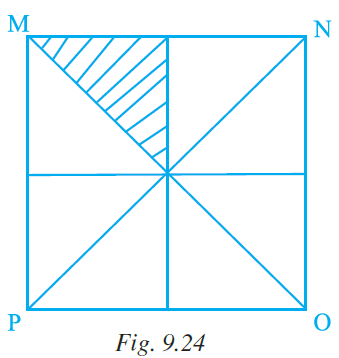Solution:-

Area of the square MNOP of Fig. 9.24 is 144 cm2. Area of each triangle is 18cm2.

From the question it is given that, Area of the square MNOP is 144 cm2.

Then, area of triangle = (1/8) × Area of the square MNOP

= 1/8 × 144

= 18 cm2

41. In Fig. 9.25, area of parallelogram BCEF is cm2 where ACDF is a rectangle.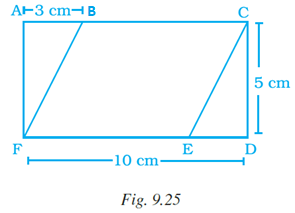Solution:-

In area of parallelogram BCEF is 35cm2 where ACDF is a rectangle.

From the figure FE = FD – AB

= 10 – 3

= 7 cm

Then, area of parallelogram = base × height

= FE × CE [CD = CE]

= FE × CD

= 7 × 5

= 35 cm2

42. To find area, any side of a parallelogram can be chosen as of the parallelogram.

Solution:-

To find area, any side of a parallelogram can be chosen as base of the parallelogram.

43. Perpendicular dropped on the base of a parallelogram from the opposite vertex is known as the corresponding of the base.

Solution:-

Perpendicular dropped on the base of a parallelogram from the opposite vertex is known as the corresponding height/altitude of the base.

44. The distance around a circle is its .

Solution:-

The distance around a circle is its circumference.

45. Ratio of the circumference of a circle to its diameter is denoted by symbol .

Solution:-

Ratio of the circumference of a circle to its diameter is denoted by symbol π.

46. If area of a triangular piece of cardboard is 90 cm2, then the length of altitude corresponding to 20 cm long base is cm.

Solution:-

If area of a triangular piece of cardboard is 90 cm2, then the length of altitude corresponding to 20 cm long base is 9cm.

We know that, area of triangle = ½ × base × height

90 = ½ × 20 × height

Height = 9 cm

47. Value of π is approximately.

Solution:-

Value of π is (22/7)=3.14 approximately.

48. Circumference ‘C’ of a circle can be found by multiplying diameter ‘d’ with .

Solution:-

Circumference ‘C’ of a circle can be found by multiplying diameter ‘d’ with π.

We know that, circumference of circle when radius is considered = 2 πr

When diameter is considered = πd

49. Circumference ‘C’ of a circle is equal to 2π × .

Solution:-

Circumference ‘C’ of a circle is equal to 2π × r.

50. 1 m2 = cm2.

Solution:-

1 m2 = 10000 cm2.

We know that, 1 m = 100 cm

1 m2 = 1002 cm2

51. 1 cm2 = mm2.

Solution:-

1 cm2 = 100 mm2.

We know that, 1 cm = 10 mm

1 cm2 = 102 mm2

52. 1 hectare = m2.

Solution:-

1 hectare = 10,000 m2

53. Area of a triangle = ½ × base × .

Solution:-

Area of a triangle = ½ × base × height.

54. 1 km2 = m2.

Solution:

1 km2 = 10,00,000 m2.

We know that, 1 km = 1000 m

1 km2 = 10002 m2

55. Area of a square of side 6 m is equal to the area of squares of each side 1 cm.

Solution:-

Area of a square of side 6 m is equal to the area of 3,60,000 squares of each side 1 cm.

Let us assume the number of squares having side of 1 cm be ‘x’.

As per the condition in the question,

Area of the side 6 m square = Area of side 1 cm square

(6m)2 = x(1cm)2

360000 cm2 = x cm2 … [because 1 m = 100 cm]

x = 360000cm2/1 cm2

x = 360000

56. 10 cm2 = m2.

Solution:-

10 cm2 = 0.001 m2.

We know that, 1 m = 100 cm

1 m2 = 10000 cm2

10 cm2 = 10/10000

= 0.001 m2

In Questions 57 to 72, state whether the statements are True or False.

57. In Fig. 9.26, perimeter of (ii) is greater than that of (i), but its area is smaller than that of (i).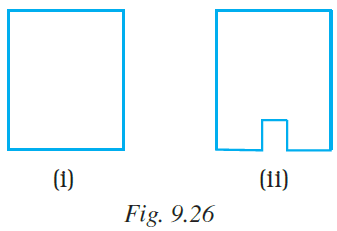Solution:-

True.

Perimeter of a closed figure is the distance around it while area is the measure of the part of plane or region enclosed by it.

Hence, perimeter of (ii) is greater than that of (i), but its area is smaller than that of (i).

58. In Fig. 9.27,

(a) area of (i) is the same as the area of (ii).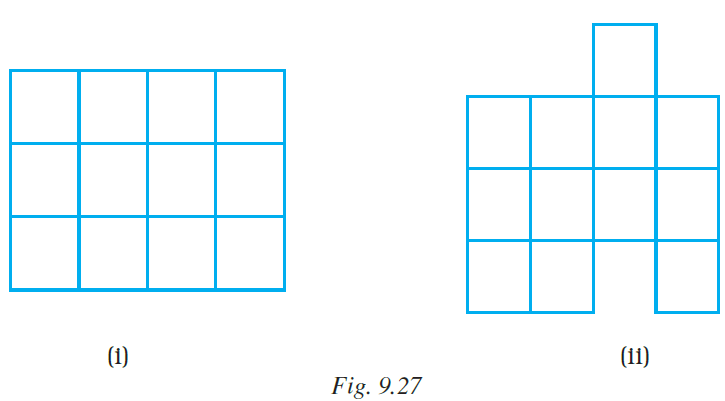Solution:-

True.

By observing the figure, we can say that area of both figures are same, because number of blocks used in both figures are same.

(b) Perimeter of (ii) is the same as (i).

Solution:-

False.

By observing the figures, we can say that there are 2 new sides added in figure (ii).

Therefore, the perimeter of (ii) is not same as (i).

(c) If (ii) is divided into squares of unit length, then its area is 13 unit squares.

Solution:-

False.

We know that, area of square = side × side

Then area of 1 square = 1 × 1

= 1 unit squares

There are 12 squares in figure (ii).

Therefore, area of figure (ii) = 12 × 1 = 12 unit squares

(d) Perimeter of (ii) is 18 units.

Solution:-

True.

The perimeter refers to the total length of the sides or edges of a polygon.

59. If perimeter of two parallelograms are equal, then their areas are also equal.

Solution:-

False.

60. All congruent triangles are equal in area.

Solution:-

True.

We know that congruent triangles have equal size and shapes.

61. All parallelograms having equal areas have same perimeters.

Solution:-

False

Now answer Questions 62 to 65:

62. Observe all the four triangles FAB, EAB, DAB and CAB as shown in Fig. 9.28:

All triangles have the same base and the same altitude.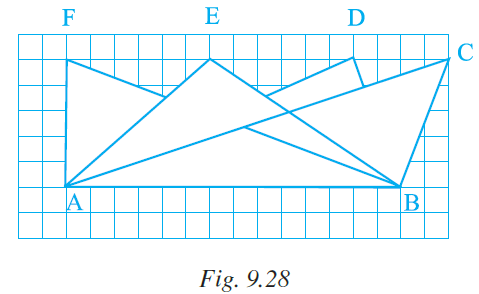Solution:-

True.

From the given figure, all triangles have the same base and the same altitude.

63. All triangles are congruent.

Solution:-

False.

64. All triangles are equal in area.

Solution:-

True.

From the given figure, all triangles have the same base and the same altitude.

65. All triangles may not have the same perimeter.

Solution:-

True.

From the given figure, we can say that all triangles may not have the same perimeter.